# 图像聚类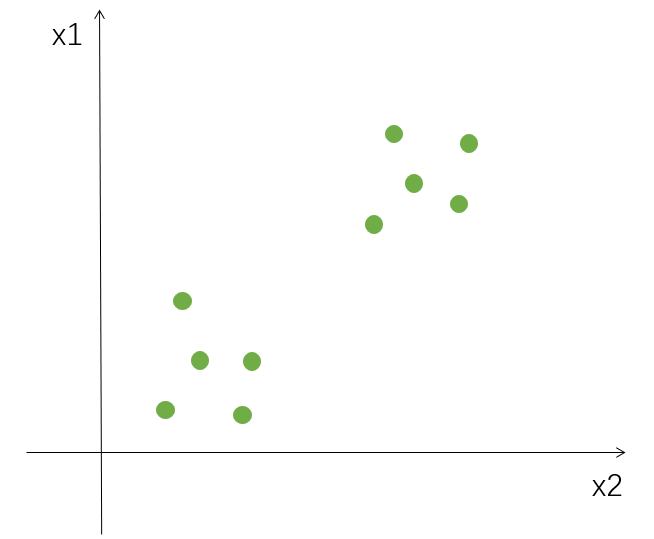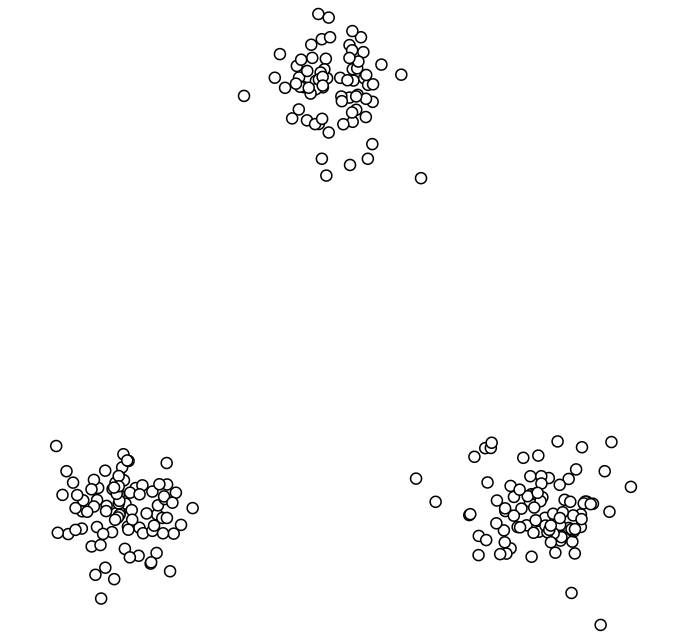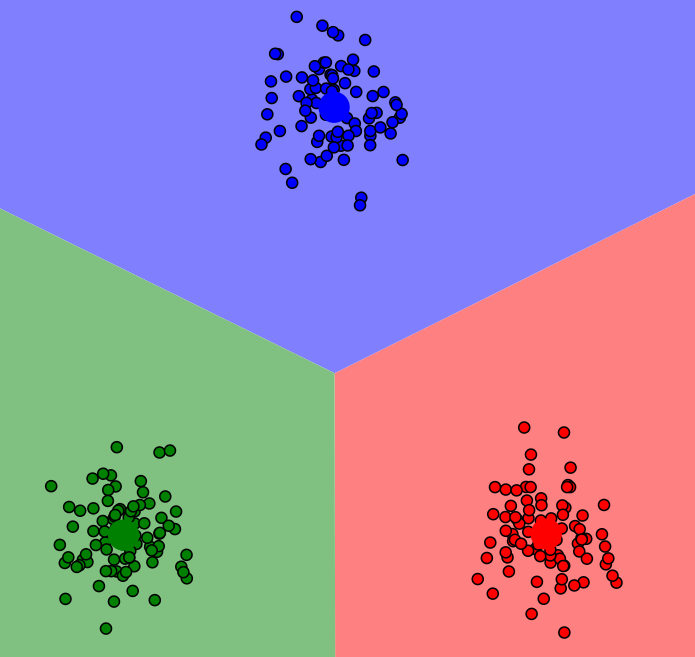## （一）K-means 聚类

1.要得到簇的个数需要指定K值（k=2，输入的数据聚成2个堆；k=3，输入的数据聚成3个堆）

2.质心：数据的均值，即各个数据在各个维取平均值得到的值（例如现在有x轴，y轴，x轴上所有数据取均值，y轴所有数据取均值，这样我们就得到了一个质心。每个簇都可以得到一个质心，质心在后面迭代的时候要用到。）

3.距离的度量：聚类简单理解就是把相似的东西聚到一起，如何判断两个样本点是不是相似的呢，这个就要根据距离做判断，最常见的计算方式就是欧几里得距离（直接算两个点的欧式距离）和余弦相似度（先标准化），当使用欧式距离的时候，先要对数据进行标准化

什么是标准化：比如现在有两个维度，x轴，y轴，x轴数据0.01,0.02,0.04，y轴数据100,200,300，当计算相似度的时候，x轴的差异无论怎么算都比较小，y轴的差异无论怎么算都比较大，那样我们潜意识里就认为相似度主要由y轴决定，实际算出来也是这样，所以说，在使用距离的度量的时候，基本情况下，都要对所有数据进行标准化，比如让x轴取值范围在0到1之间，y轴取值范围在0到1之间，让数据x和y基本在一个比较小的范围内浮动，比如说都是0到1，或者-1到1。

4.优化目标：

$min\sum_{i=1}^{k}\sum_{x\in C_{i}}^{}dist(c_{i},x)^{2}$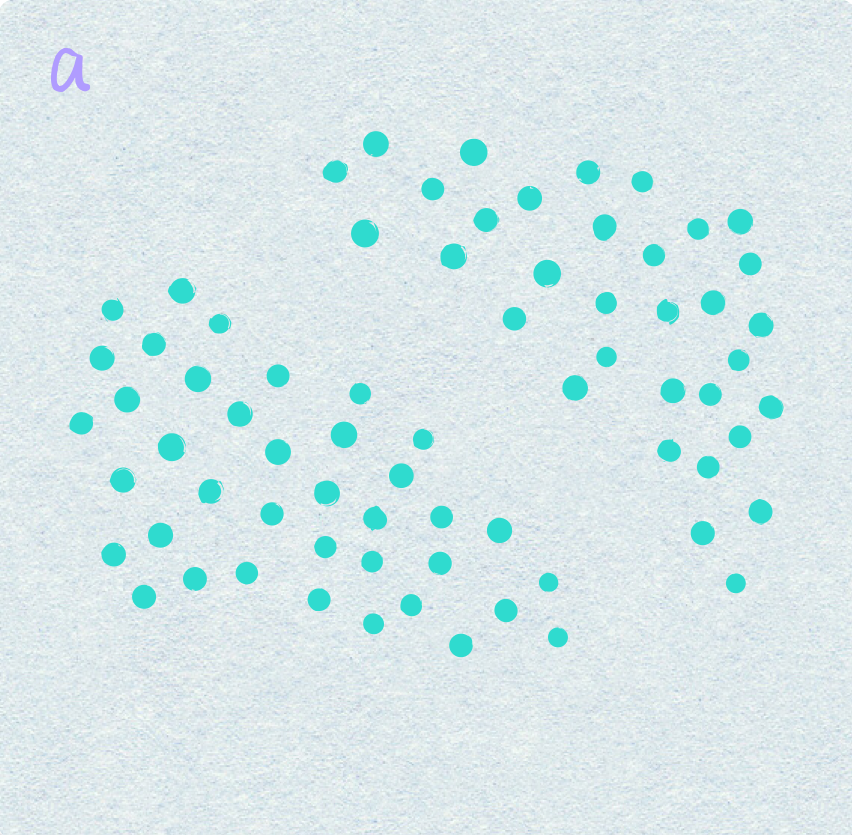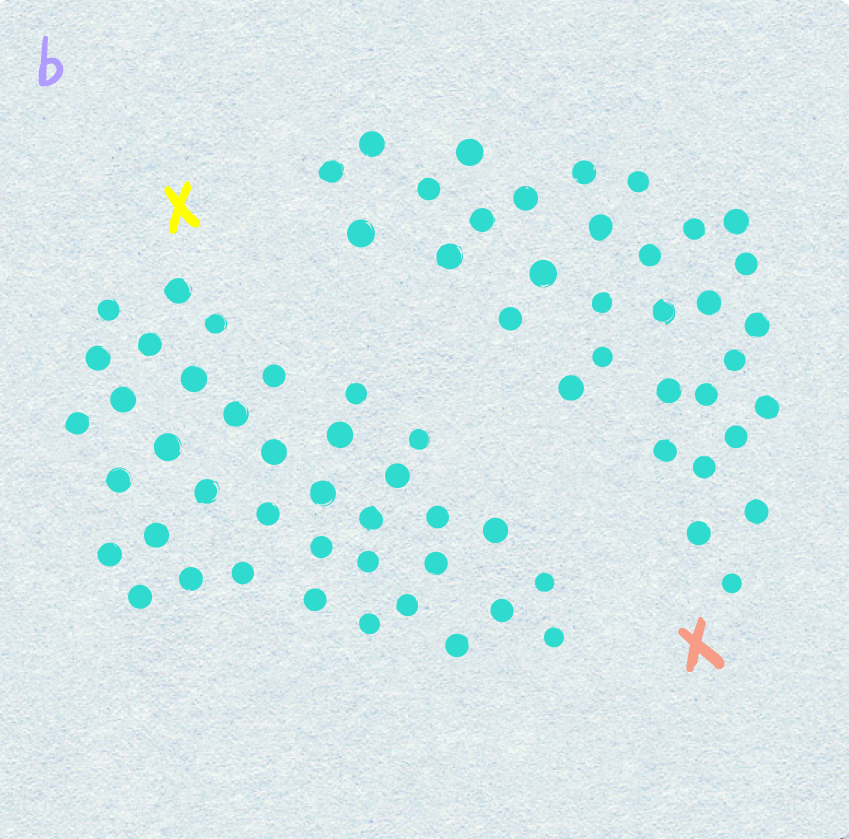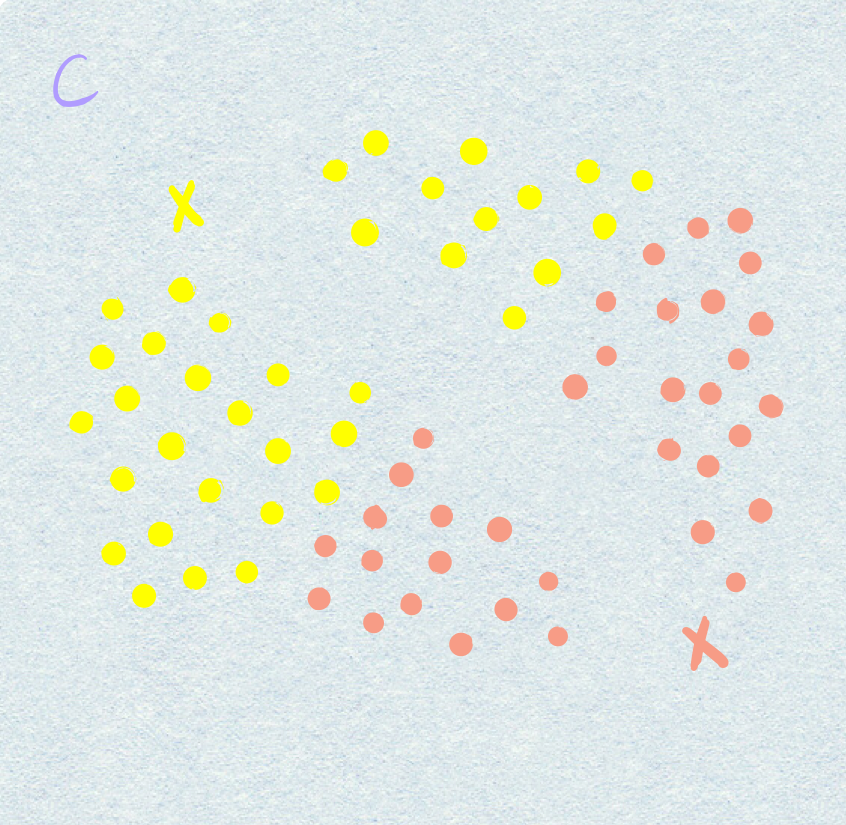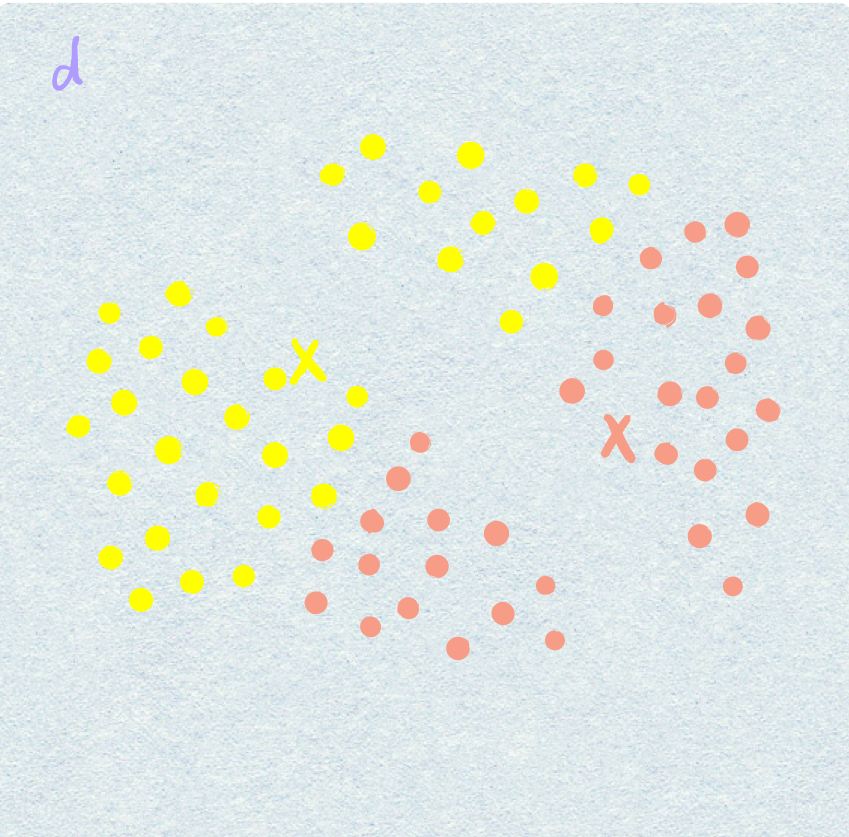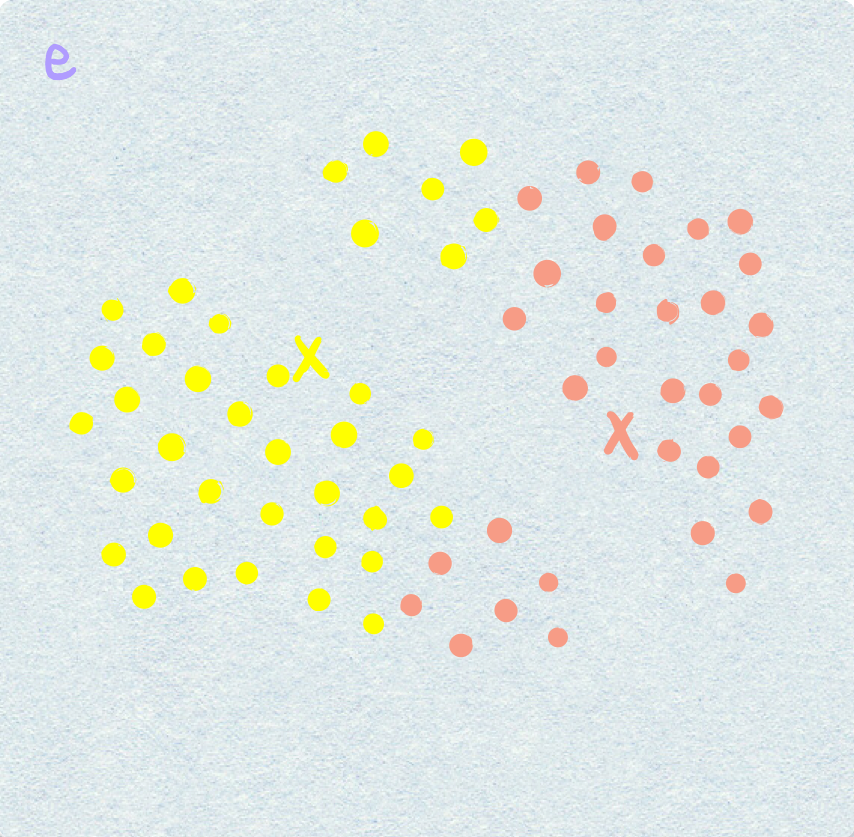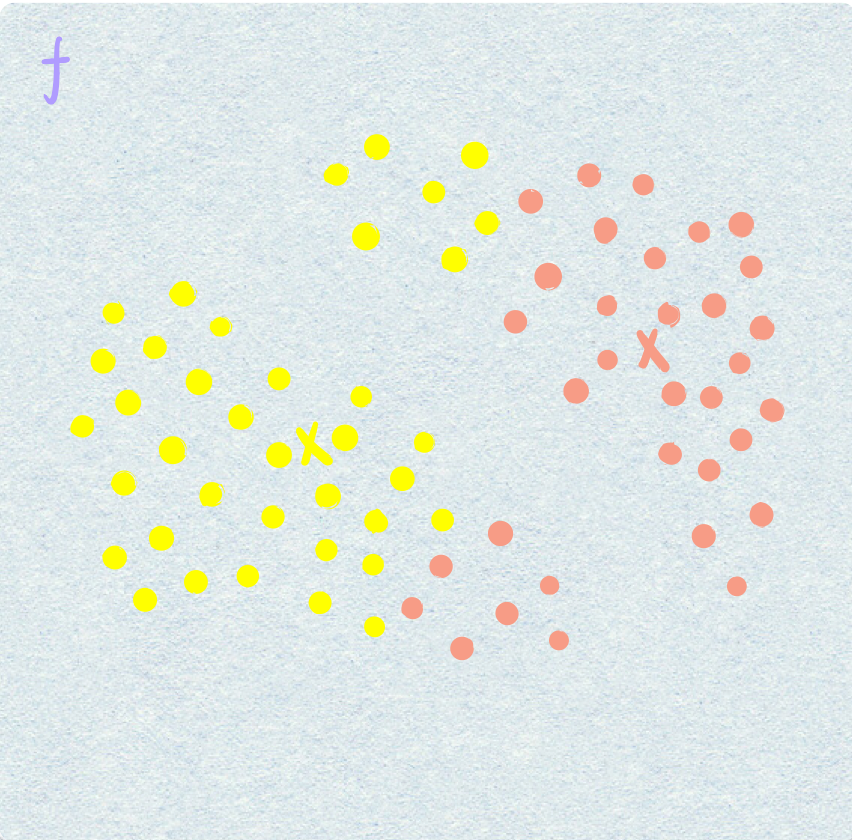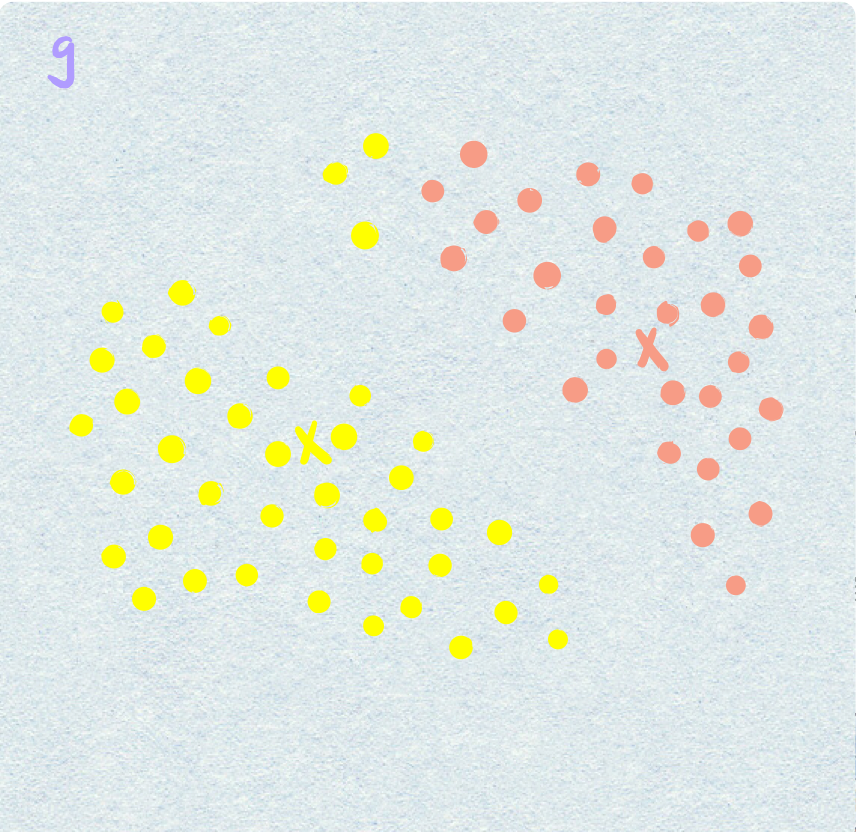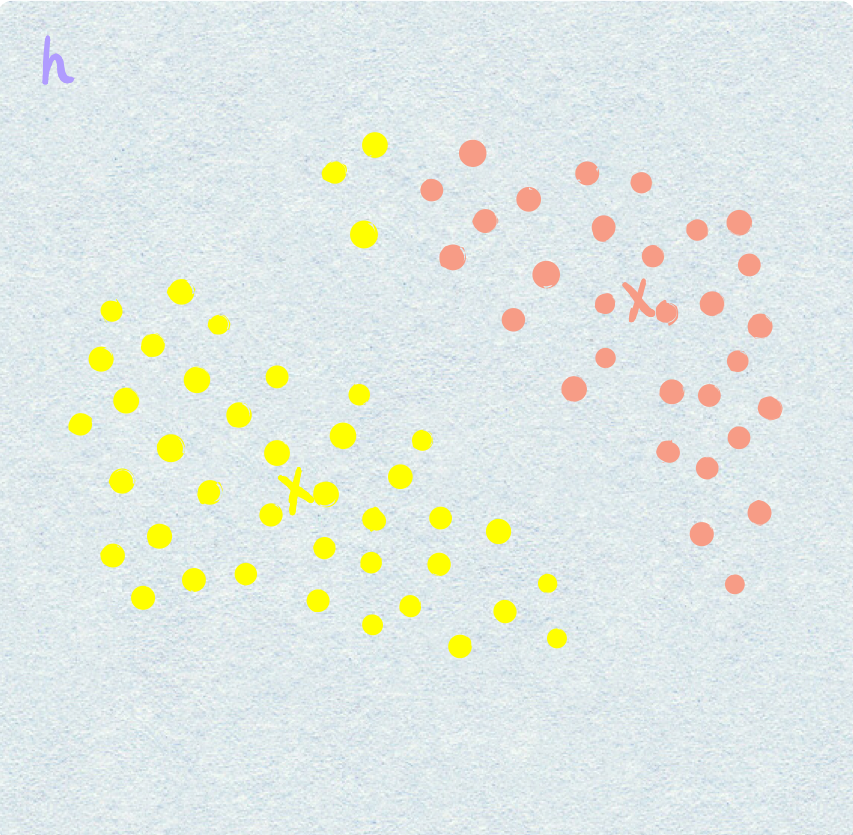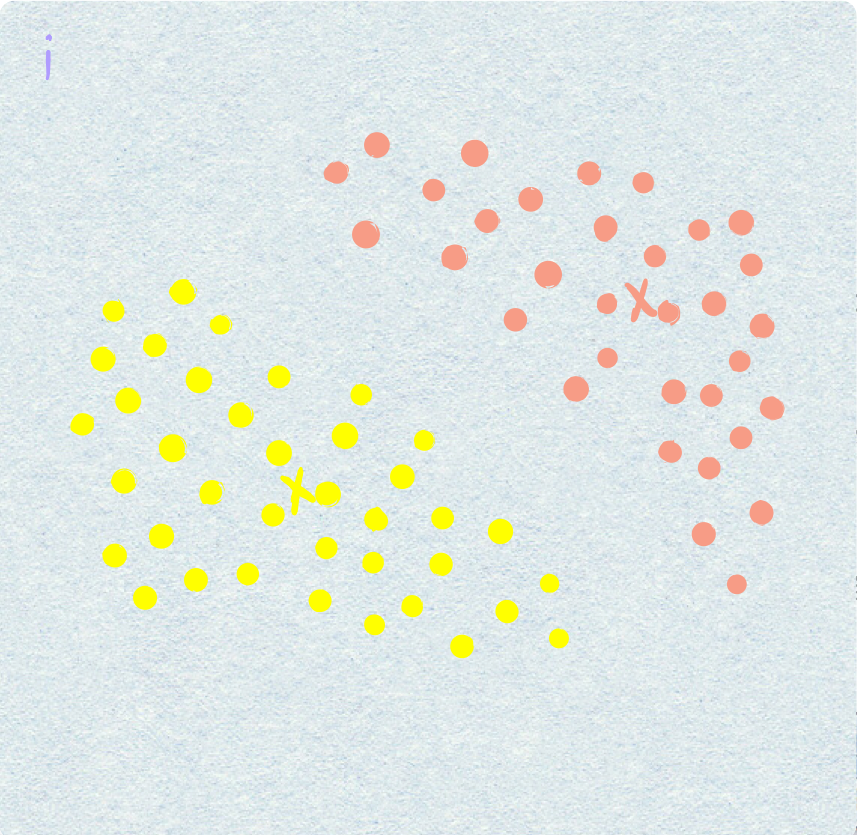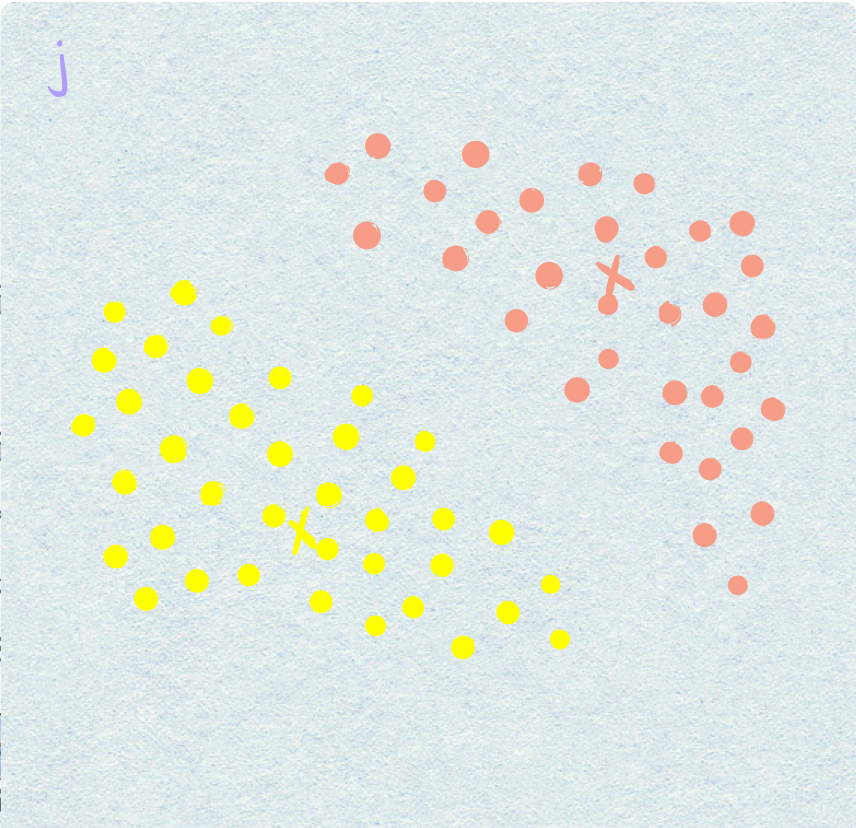1. 由于是无监督问题，不知道每一个点该属于哪一个簇，假设k=2（ k=2,分两堆;k=3,分三堆，此处以k=2举例 ），首先会在(a)图中初始化两个点。
2. 如(b)图所示，一个黄色的，一个粉色的，这两个点是随机初始化的。然后基于初始化的这两个点，算每一个其他的点是属于粉色还是黄色，如何判断，就是算这个点到黄色点的距离（如下图），假设为$d_1$，这个点到红色点的距离，假设为$d_2$$d_1$<$d_2$，认为当前点属于黄色，因为距离越小越相似。以此类推。把每一个样本点都算一遍。一些离黄色近的化成黄色，一些离粉色近的化成粉色。得到了( c )图。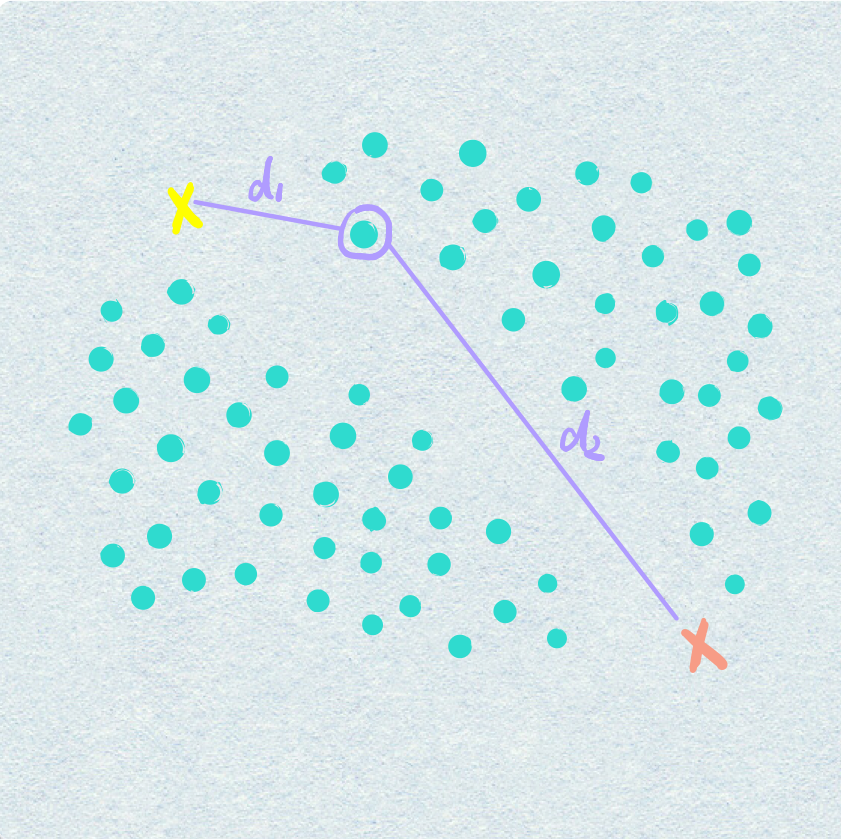3. 可以看到c图离预期效果还有一段距离。所以要进行更新，更新衡量的依据，也就是两个质心，因为这两个质心是随机选择的，肯定是不太准的。如何更新，就是把所有黄色的点拿出来，重新算质心，所有粉色的点同样算质心。得到了(d)图。
4. 质心更新完之后，重新遍历样本中所有的点，比如原来黄色的点到粉色质心的距离$d_2$更小（如下图），现在就更新到粉色。以此类推。得到了(e)图。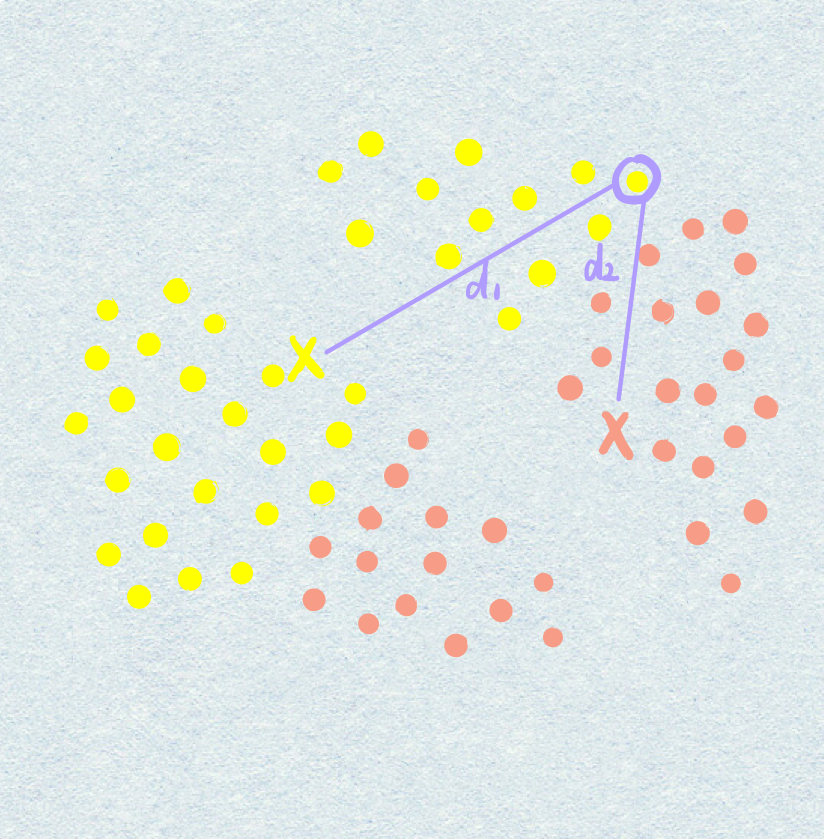5. 再按照之前的套路，更新质心，得到(f)图。
6. 再进行遍历，找每个点的归属，然后不断的更新下去，直到更新到某一步，所有的样本点不再发生变化。不再发生变化，更新基本结束，因为再更新还是原来状态，黄色的还是属于黄色，粉色的还是属于粉色。

1. 简单（原理很简单，理解起来也很简单）
2. 快速（直接考虑k值就可以了）
3. 适合常规数据集（比如上图，两堆看起来就能分开）

1. k值难确定（当拿到一个数据，可以做可视化展示，但是由于标签不知道是什么，所以不知道究竟分成几个簇，普遍情况下需要设置多组，然后看效果）。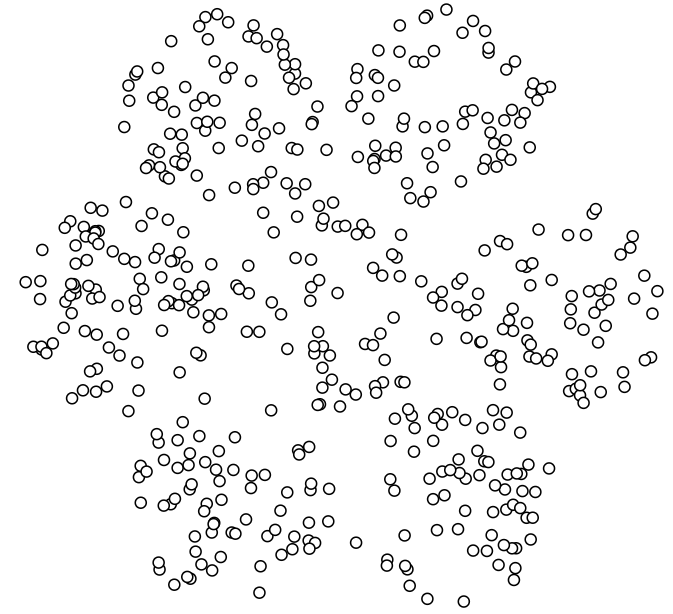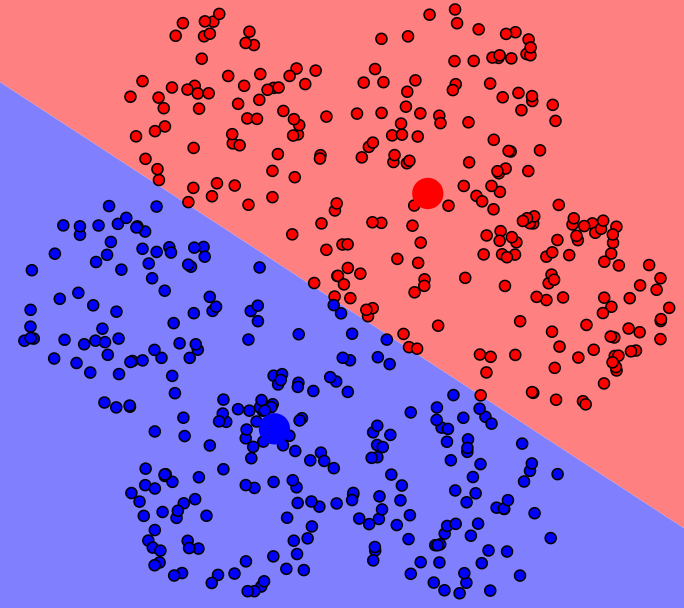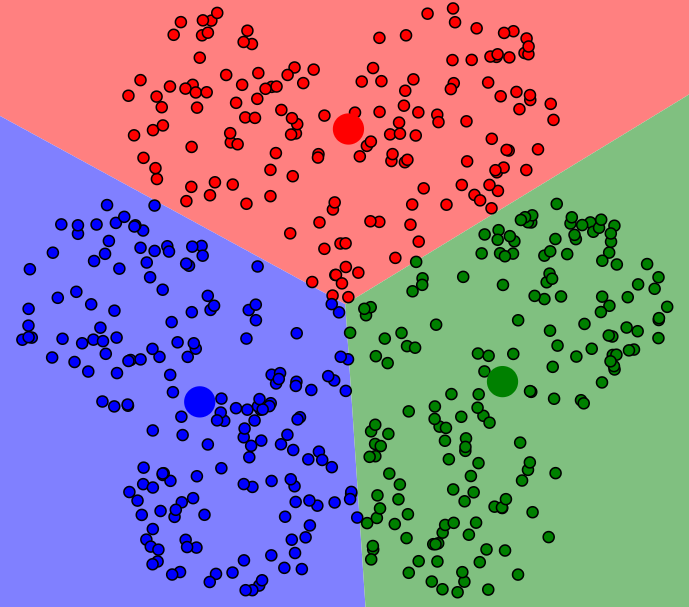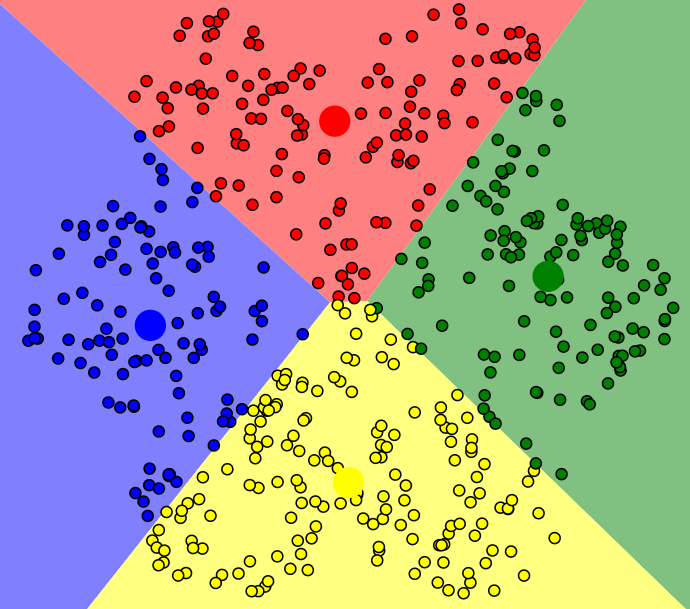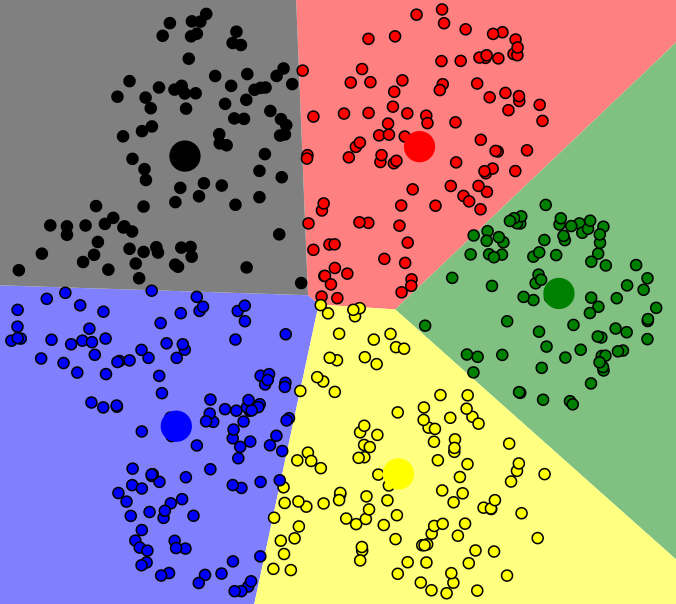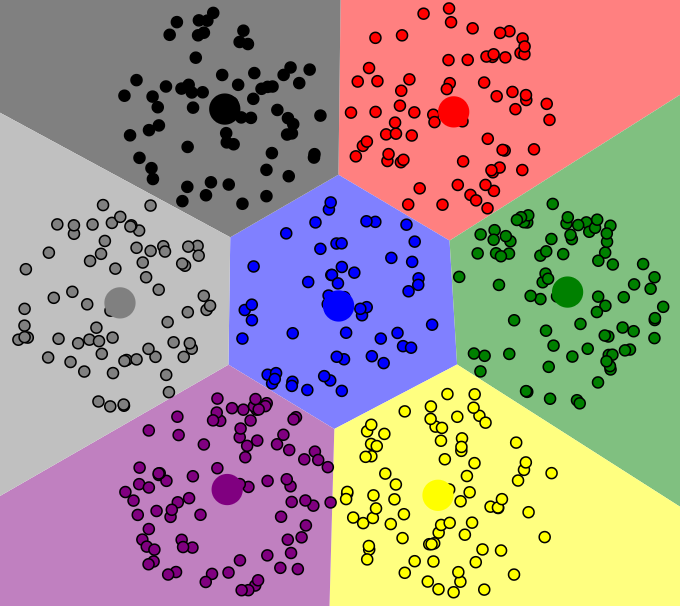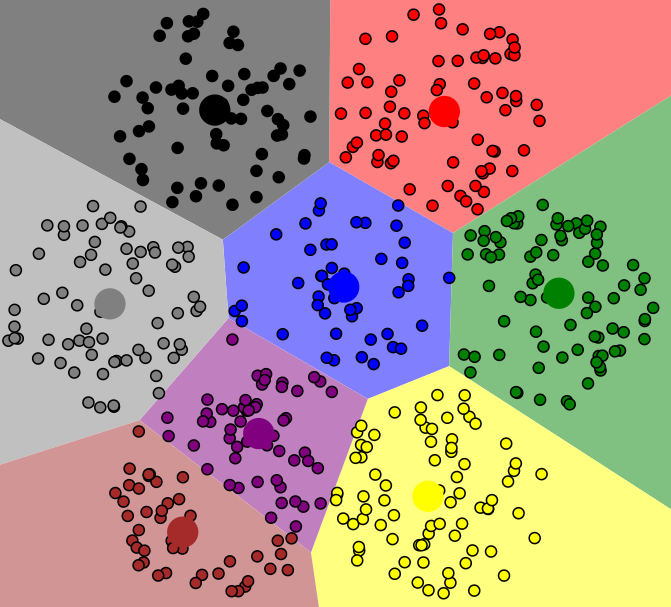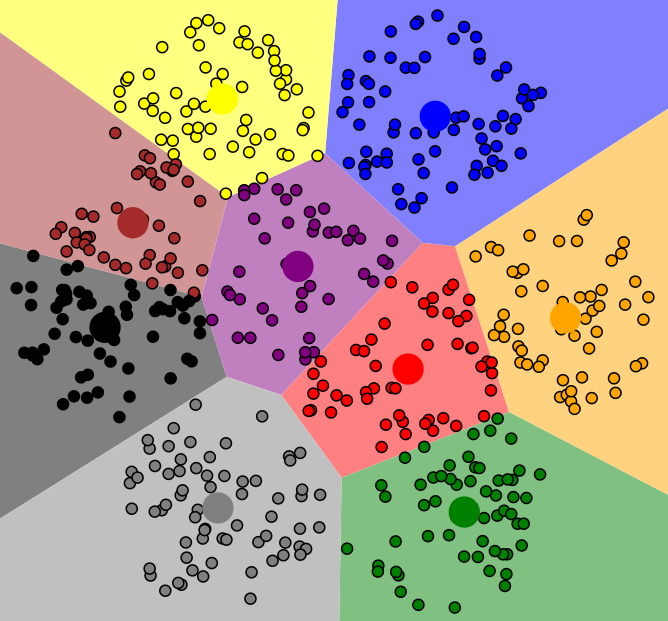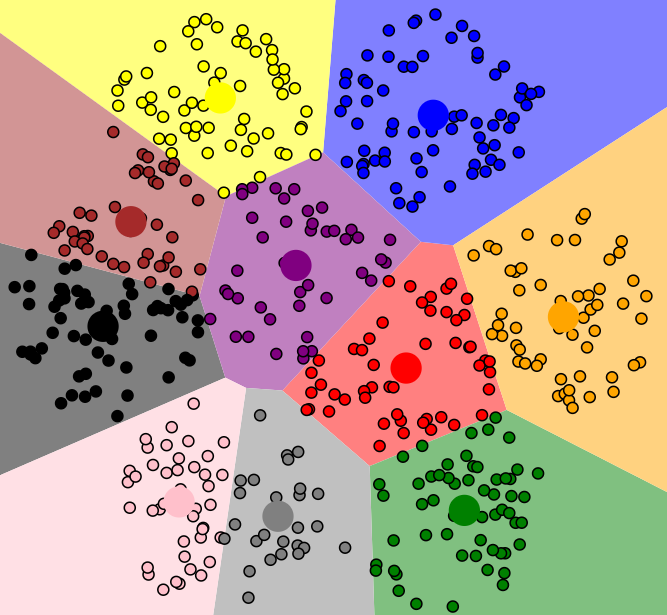可以看到，好像分成几个簇都能划分出来，所以到底分成多少个簇合适，这个比较难确定。（小插曲：可能会有疑惑，为什么第八张图和第九张图与前面的分布有点不一样，是因为博主有点强迫症哈，本来想用第七张图与第六章图对比一下就可以了，结果发现七张图不好排版，但是这时候博主已经关掉了这个页面，所以第二次运行，又会重新设置初值，不同的初值得到的结果是不一样的，算是误打误撞发现新大陆吧）
2. 复杂度与样本呈现线性相关（复杂度与样本个数相关，所有的样本都要和质心计算，如果与样本过多，计算越多，复杂度就相当高了）
3. 很难发现任意形状的簇（并不能保证一个数据是一个非常规整的簇，举例，如下图，可以认为里面是一个簇，外面是一个簇，但是用K-means算法没办法把里面和外面分开，而是会分为左边一个簇，右边一个簇）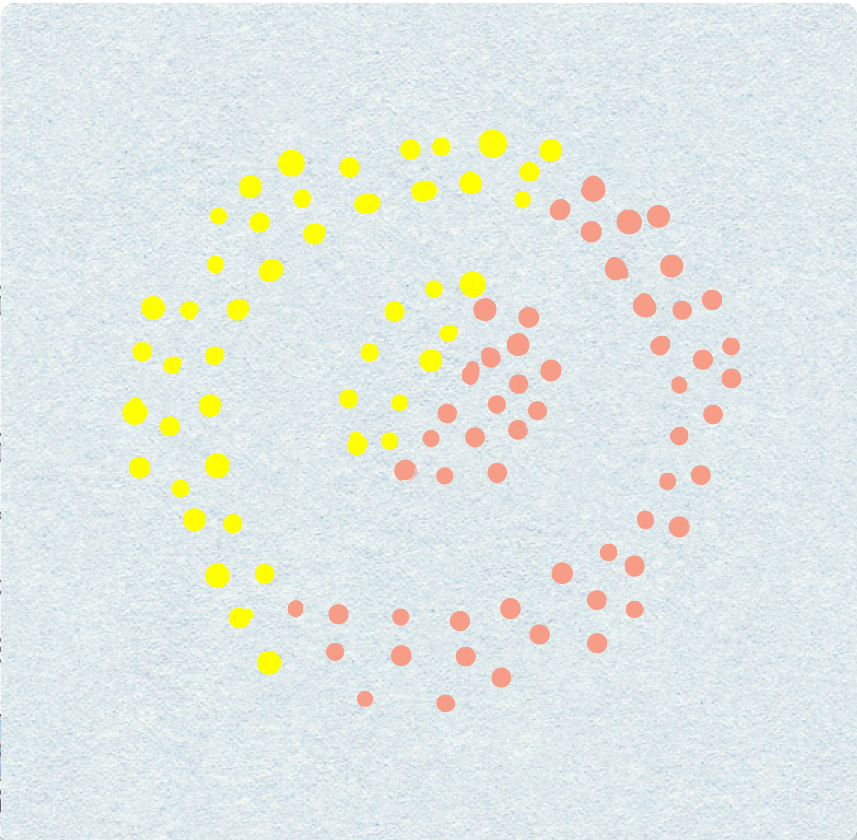下面来验证一下，这是一个笑脸，理想情况下应该分成两个眼睛，一个嘴巴，一个圆圈脸，四个簇，但是看下运行效果：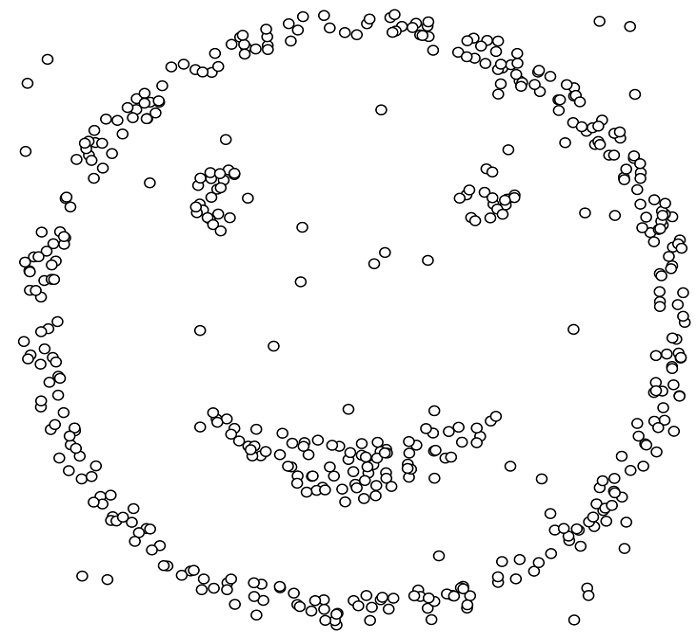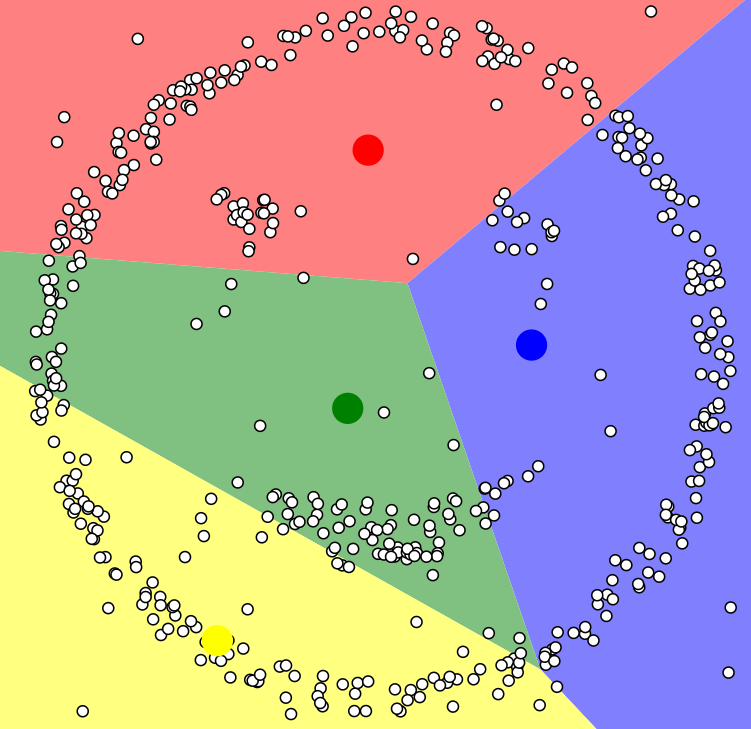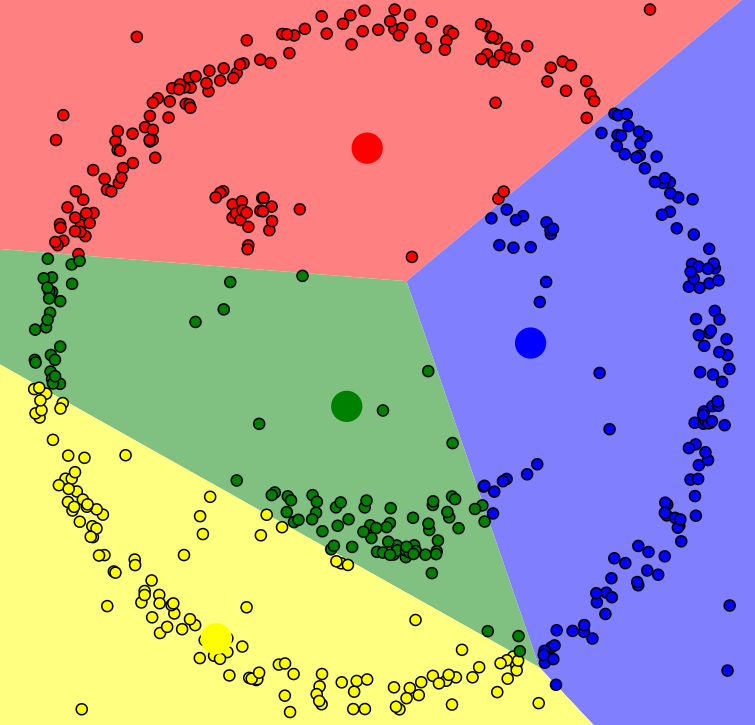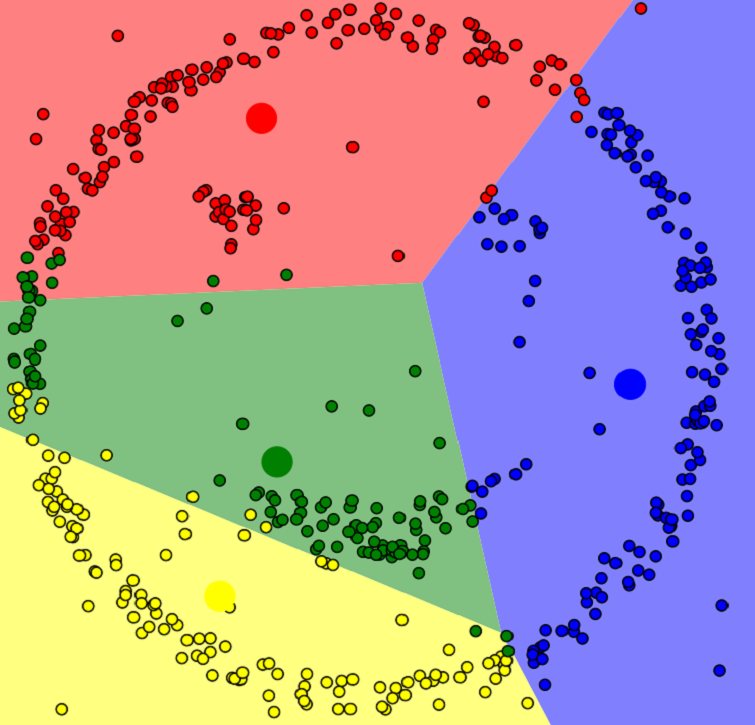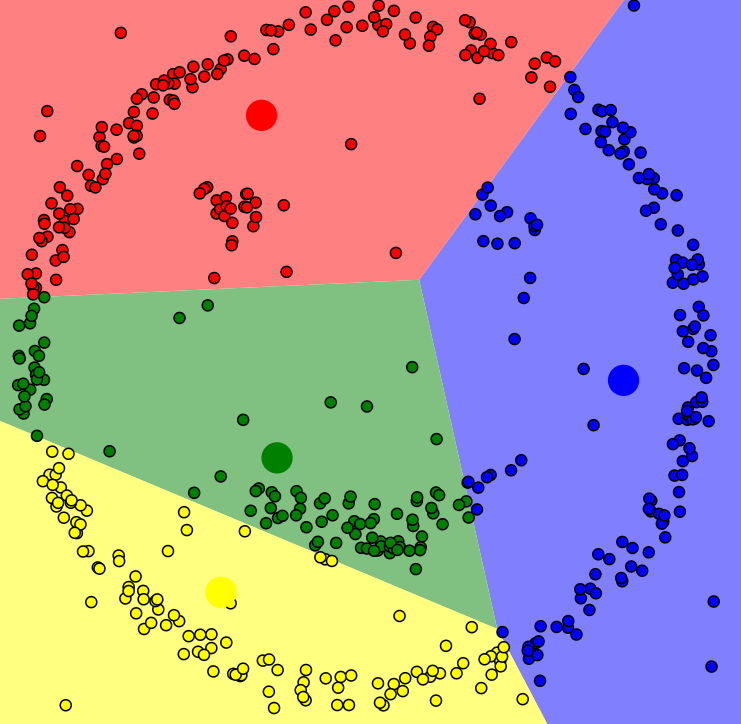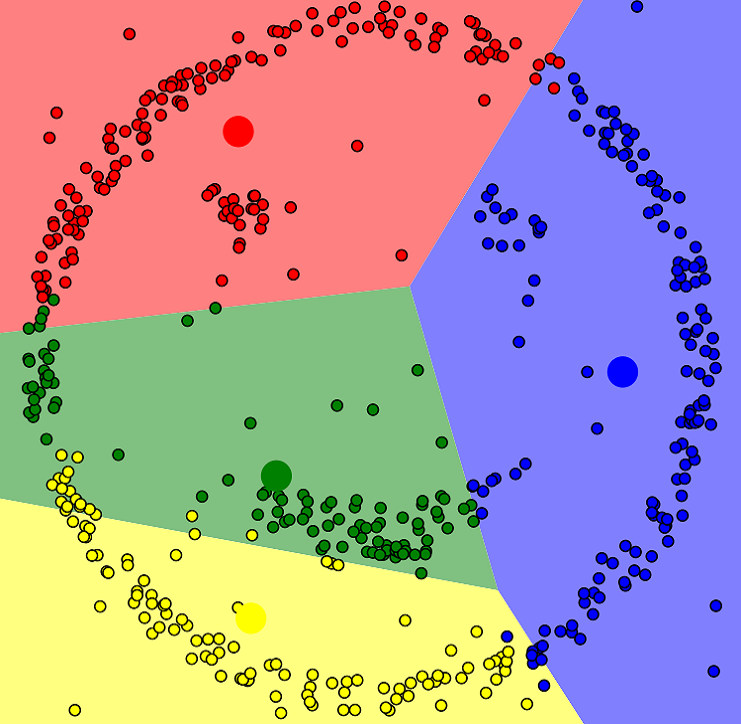一直迭代，最后得到结果：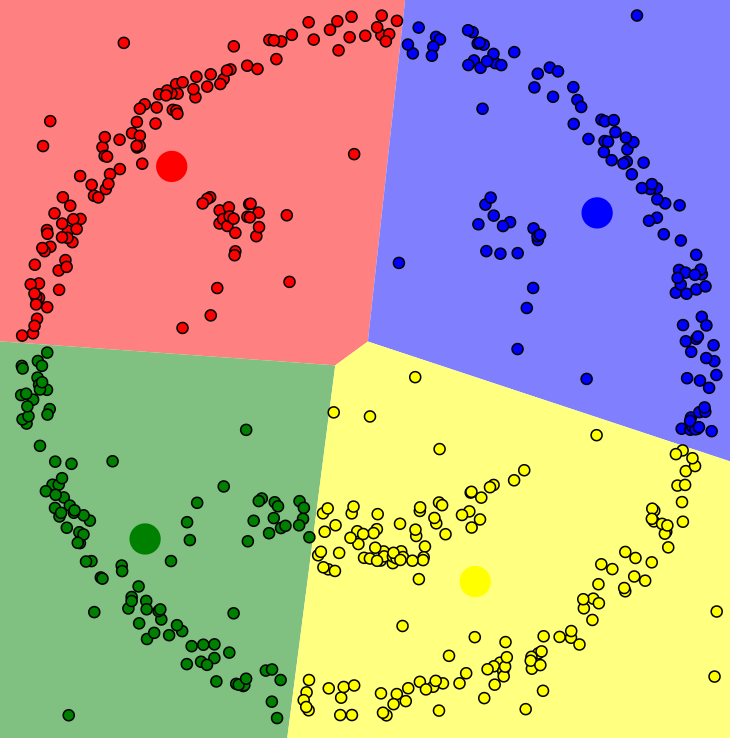# beer dataset
import pandas as pd
beer = pd.read_csv('data.txt', sep=' ')   #导入数据，这是20个啤酒数据，包括四个属性
beer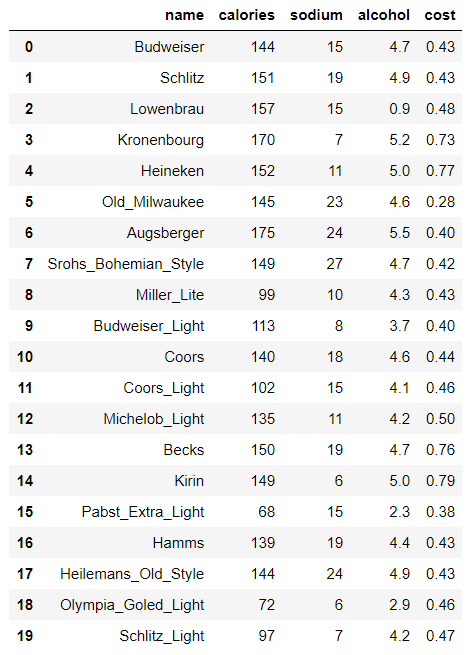X = beer[["calories","sodium","alcohol","cost"]]   #聚类算法的输入是x，x是所有的特征，聚类算法不需要构建标签

from sklearn.cluster import KMeans
km = KMeans(n_clusters=3).fit(X)     #用三个堆做聚类
km2 = KMeans(n_clusters=2).fit(X)    #用两个堆做聚类

km.labels_          #显示每个数据属于哪个类别，三个堆，所以有0,1,2


array([0, 0, 0, 0, 0, 0, 0, 0, 1, 1, 0, 1, 0, 0, 0, 2, 0, 0, 2, 1])

km2.labels_         #两个堆，所以有0,1


array([1, 1, 1, 1, 1, 1, 1, 1, 0, 0, 1, 0, 1, 1, 1, 0, 1, 1, 0, 0])

beer['cluster'] = km.labels_
beer['cluster2'] = km2.labels_
beer.sort_values('cluster')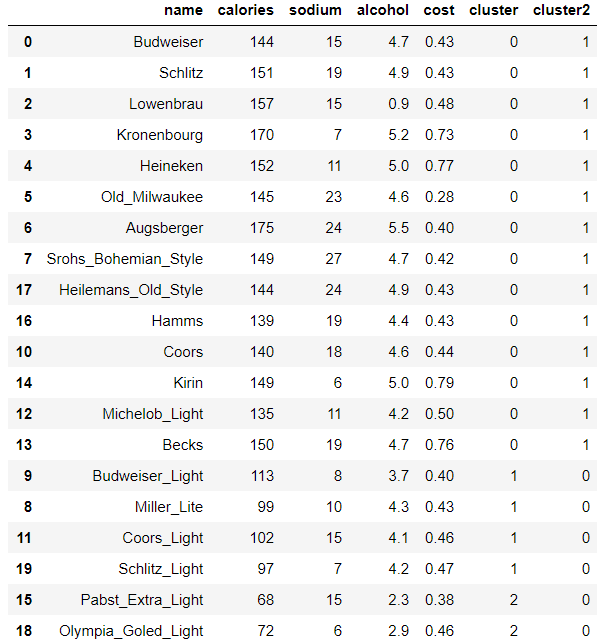from pandas.tools.plotting import scatter_matrix
%matplotlib inline
cluster_centers = km.cluster_centers_
cluster_centers_2 = km2.cluster_centers_
beer.groupby("cluster").mean()  #分别算三个cluster的均值，观察不同类别上的差异性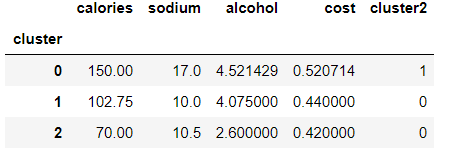beer.groupby("cluster2").mean()   #分别算三个cluster的均值，观察不同类别上的差异性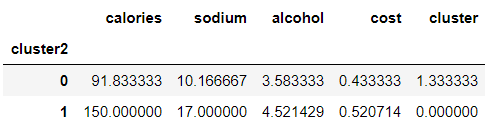centers = beer.groupby("cluster").mean().reset_index()    #计算中心点
%matplotlib inline
import matplotlib.pyplot as plt
plt.rcParams['font.size'] = 14
import numpy as np
colors = np.array(['red', 'green', 'blue', 'yellow'])   #指定绘图颜色
plt.scatter(beer["calories"], beer["alcohol"],c=colors[beer["cluster"]])

plt.scatter(centers.calories, centers.alcohol, linewidths=3, marker='+', s=300, c='black')#中心点

plt.xlabel("Calories")
plt.ylabel("Alcohol") #展示卡路里和酒精两个维度上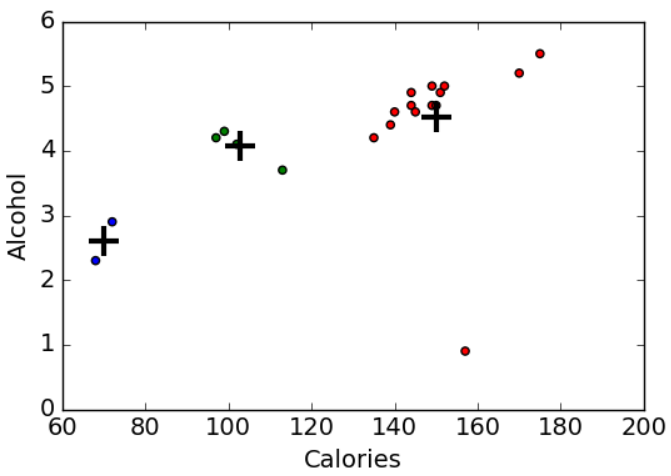scatter_matrix(beer[["calories","sodium","alcohol","cost"]],s=100, alpha=1, c=colors[beer["cluster"]], figsize=(10,10))
plt.suptitle("With 3 centroids initialized")   ##展示四个维度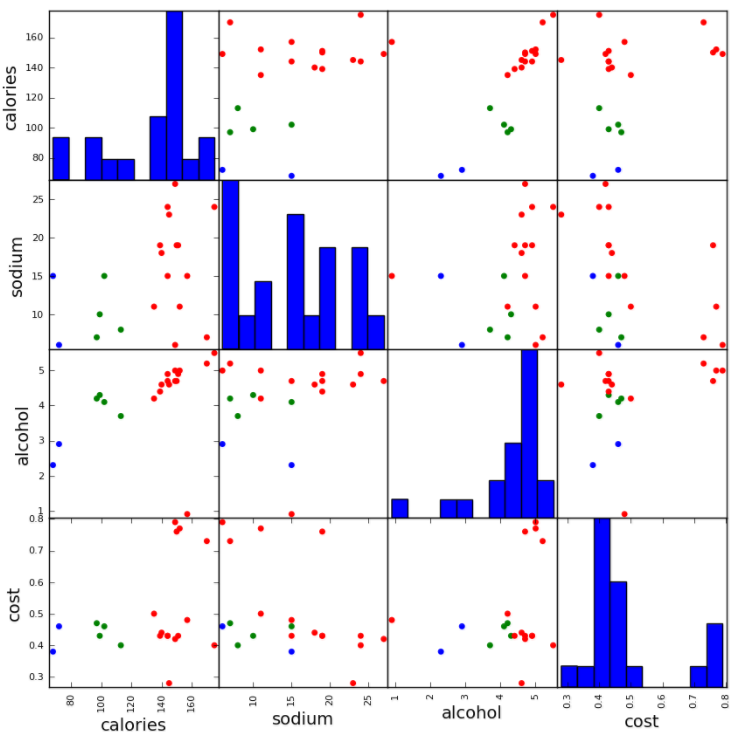from sklearn.preprocessing import StandardScaler
scaler = StandardScaler()
X_scaled = scaler.fit_transform(X)
X_scaled          #标准化，使数据更规整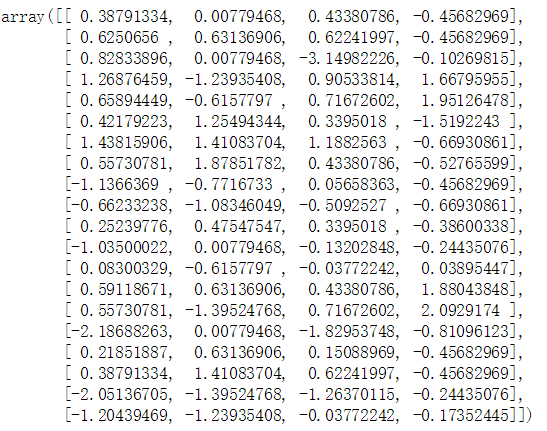km = KMeans(n_clusters=3).fit(X_scaled)
beer["scaled_cluster"] = km.labels_
beer.sort_values("scaled_cluster")   #标准化后得到的结果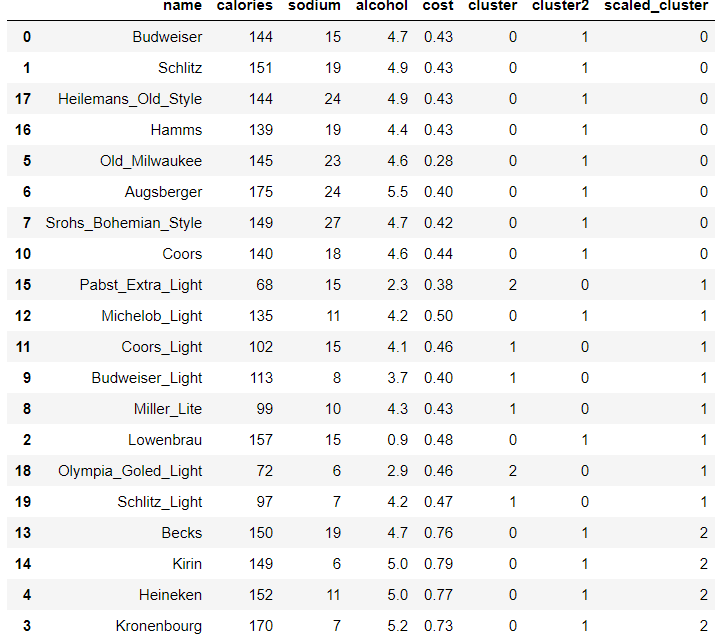beer.groupby("scaled_cluster").mean()   #分别算归一化后三个cluster的均值，观察不同类别上的差异性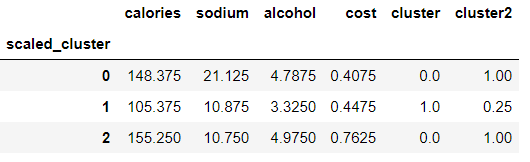pd.scatter_matrix(X, c=colors[beer.scaled_cluster], alpha=1, figsize=(10,10), s=100)  #显示四个维度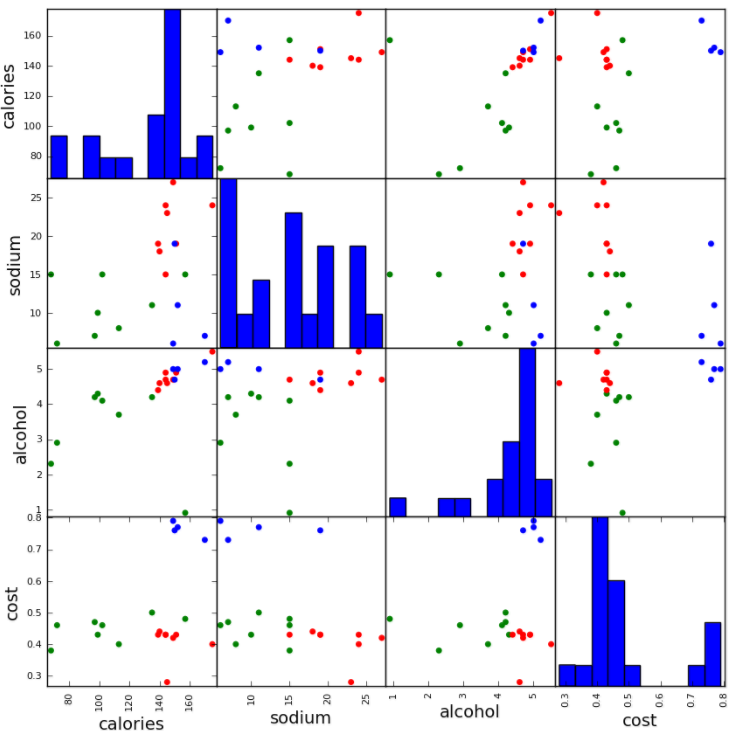$s(i)=\frac{b(i)-a(i)}{max\left \{ a(i),b(i) \right \}}$

$s(i)=\left\{\begin{matrix} 1-\frac{a(i)}{b(i)} & a(i)<b(i)\\ 0& a(i)=b(i)\\ \frac{b(i)}{a(i)}-1 & a(i)>b(i) \end{matrix}\right.$

1. 计算样本i到同簇其他样本的平均距离a(i)。a(i)越小，说明样本i越应该被聚类到该簇。将a(i)称为样本i的簇内不相似度。
2. 计算样本i到其他某簇Cj 的所有样本的平均距离bij，称为样本i与簇Cj 的不相似度。定义为样本i的簇间不相似度：b(i) =min{bi1, bi2, …, bik}，b(i)越大越好，越大说明当前样本越不可能被分到其他簇。
3. si接近1，则说明样本i聚类合理。（ b(i)-a(i)理想情况下等于b(i)，max{a(i),b(i)}理想情况下等于b(i) ）
4. si接近-1，则说明样本i更应该分类到另外的簇
5. 若si 近似为0，则说明样本i在两个簇的边界上。
from sklearn import metrics
score = metrics.silhouette_score(X,beer.cluster)#不做归一化得到的结果 si值
score_scaled = metrics.silhouette_score(X,beer.scaled_cluster)#归一化之后得到的结果 si值
print(score_scaled, score)#发现做完归一化结果反而低了，说明归一化有时候并不能使结果变好，比如某些特征确实很重要，那做完归一化就消除了差异


0.673177504646 0.179780680894

scores = []
for k in range(2,20):    #遍历K值，看效果
labels = KMeans(n_clusters=k).fit(X).labels_
score = metrics.silhouette_score(X, labels)
scores.append(score)

scores   #选择轮廓系数比较高的比较合适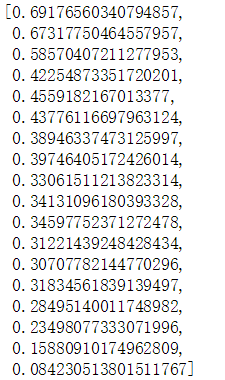plt.plot(list(range(2,20)), scores) #画图更直观展示
plt.xlabel("Number of Clusters Initialized")
plt.ylabel("Sihouette Score")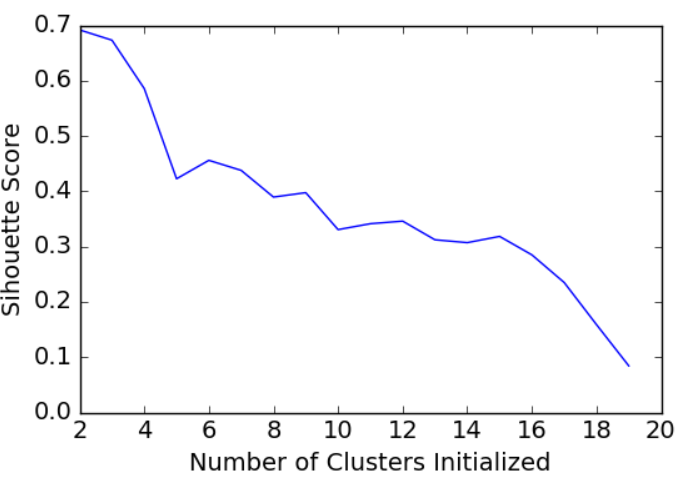# -*- coding: utf-8 -*-
# 导包
from skimage import io
from sklearn.cluster import KMeans
import numpy as np

# 读取图片

# 显示图片
io.imshow(image)

# 获取图片压缩前的信息
print ('image的类型：', type(image))  # numpy.ndarray类型
print ('image的尺寸：', image.shape)  # 显示尺寸
print ('image的宽度：', image.shape)  # 图片宽度
print ('image的高度：', image.shape)  # 图片高度
print ('image的通道数：', image.shape)  # 图片通道数
print ('image的总像素个数：', image.size)  # 显示总像素个数
print ('image的最大像素值：', image.max())  # 最大像素值
print ('image的最小像素值：', image.min())  # 最小像素值
print ('image的像素平均值：', image.mean())  # 像素平均值

# rows*cols*channel = 482*500*3
rows = image.shape
cols = image.shape
channel = image.shape

# 样本数*channel = 241000*3
# 每个样本在不同通道都有存在1个点，即此时每个样本对应于3个点
image = image.reshape(image.shape * image.shape, channel)

# 样本数*channel = 241000*1
# 使用Kmeans算法将3通道变为1通道（将原来很多的颜色用少量的颜色来表示），即此时每个样本只存在1个点
# n_clusters：K值，把集合分成K个簇；n_init：指定CPU个数；max_iter：最大的迭代次数
kmeans = KMeans(n_clusters=128, n_init=10, max_iter=200)
kmeans.fit(image)

clusters = np.asarray(kmeans.cluster_centers_, dtype=np.uint8)
# labels_：每个点的标签
labels = np.asarray(kmeans.labels_, dtype=np.uint8)
# rows*cols*channel = 482*500*1
labels = labels.reshape(rows, cols)

np.save('D:\\Python\\chapter6\\codebook_test.npy', clusters)
# 保存压缩后的图片
io.imsave('D:\\Python\\chapter6\\compressed_test.jpg', labels)
# 读取压缩后的图片

# 获取图片压缩后的信息
print ('newimage的类型：', type(newimage))  # numpy.ndarray类型
print ('newimage的尺寸：', newimage.shape)  # 显示尺寸
print ('newimage的宽度：', newimage.shape)  # 图片宽度
print ('newimage的高度：', newimage.shape)  # 图片高度
# print ('newimage的通道数：', newimage.shape)  #图片通道数
print ('newimage的总像素个数：', newimage.size)  # 显示总像素个数
print ('newimage的最大像素值：', newimage.max())  # 最大像素值
print ('newimage的最小像素值：', newimage.min())  # 最小像素值
print ('newimage的像素平均值：', newimage.mean())  # 像素平均值

# 显示压缩后的图片
io.imshow(newimage)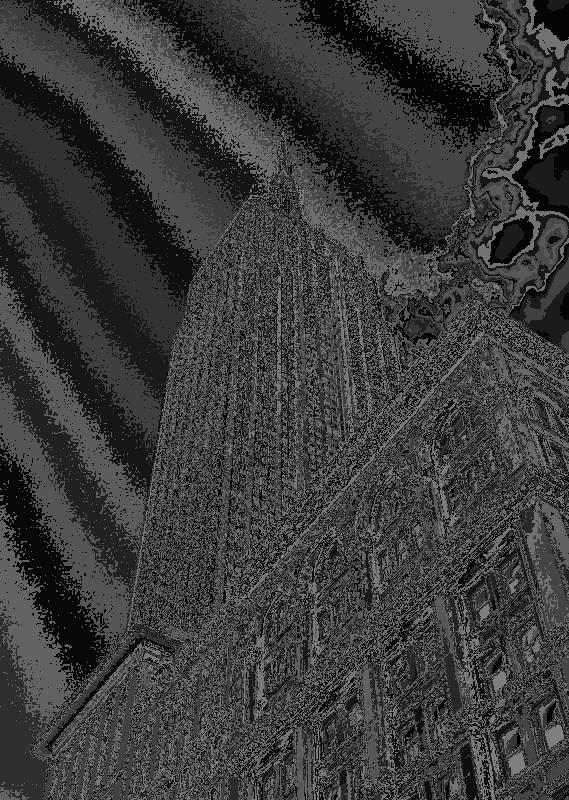(‘image\xe7\x9a\x84\xe7\xb1\xbb\xe5\x9e\x8b\xef\xbc\x9a’, <type ‘numpy.ndarray’>)
(‘image\xe7\x9a\x84\xe5\xb0\xba\xe5\xaf\xb8\xef\xbc\x9a’, (800L, 569L, 3L))
(‘image\xe7\x9a\x84\xe5\xae\xbd\xe5\xba\xa6\xef\xbc\x9a’, 800L)
(‘image\xe7\x9a\x84\xe9\xab\x98\xe5\xba\xa6\xef\xbc\x9a’, 569L)
(‘image\xe7\x9a\x84\xe9\x80\x9a\xe9\x81\x93\xe6\x95\xb0\xef\xbc\x9a’, 3L)
(‘image\xe7\x9a\x84\xe6\x80\xbb\xe5\x83\x8f\xe7\xb4\xa0\xe4\xb8\xaa\xe6\x95\xb0\xef\xbc\x9a’, 1365600)
(‘image\xe7\x9a\x84\xe6\x9c\x80\xe5\xa4\xa7\xe5\x83\x8f\xe7\xb4\xa0\xe5\x80\xbc\xef\xbc\x9a’, 255)
(‘image\xe7\x9a\x84\xe6\x9c\x80\xe5\xb0\x8f\xe5\x83\x8f\xe7\xb4\xa0\xe5\x80\xbc\xef\xbc\x9a’, 0)
(‘image\xe7\x9a\x84\xe5\x83\x8f\xe7\xb4\xa0\xe5\xb9\xb3\xe5\x9d\x87\xe5\x80\xbc\xef\xbc\x9a’, 132.4720262155829)
(‘newimage\xe7\x9a\x84\xe7\xb1\xbb\xe5\x9e\x8b\xef\xbc\x9a’, <type ‘numpy.ndarray’>)
(‘newimage\xe7\x9a\x84\xe5\xb0\xba\xe5\xaf\xb8\xef\xbc\x9a’, (800L, 569L))
(‘newimage\xe7\x9a\x84\xe5\xae\xbd\xe5\xba\xa6\xef\xbc\x9a’, 800L)
(‘newimage\xe7\x9a\x84\xe9\xab\x98\xe5\xba\xa6\xef\xbc\x9a’, 569L)
(‘newimage\xe7\x9a\x84\xe6\x80\xbb\xe5\x83\x8f\xe7\xb4\xa0\xe4\xb8\xaa\xe6\x95\xb0\xef\xbc\x9a’, 455200)
(‘newimage\xe7\x9a\x84\xe6\x9c\x80\xe5\xa4\xa7\xe5\x83\x8f\xe7\xb4\xa0\xe5\x80\xbc\xef\xbc\x9a’, 167)
(‘newimage\xe7\x9a\x84\xe6\x9c\x80\xe5\xb0\x8f\xe5\x83\x8f\xe7\xb4\xa0\xe5\x80\xbc\xef\xbc\x9a’, 0)
(‘newimage\xe7\x9a\x84\xe5\x83\x8f\xe7\xb4\xa0\xe5\xb9\xb3\xe5\x9d\x87\xe5\x80\xbc\xef\xbc\x9a’, 60.489773725834795)

DBSCAN聚类

ϵ-邻域的距离阈值：设定的半径r（DBSCAN算法需要指定两个参数，一个是半径，一个是阈值，不需要设置k值，究竟聚成多少堆，由算法决定）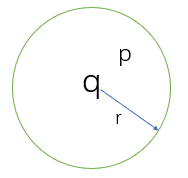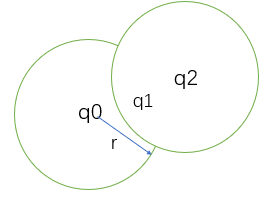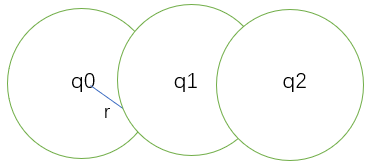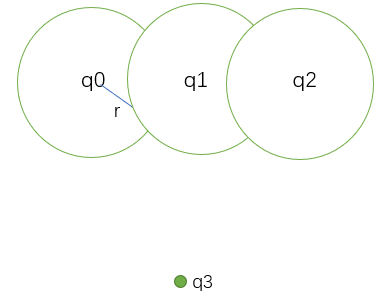标记所有对象为unvisited
Do
随机选择一个 unvited 对象 p；
标记 p 为visited；
if p 的 ϵ-（半径范围内） 领域内至少有 MinPts 个对象    #如果p是核心对象
创建一个新簇 C，并把p添加到C；
令 N 为 p 的 ϵ- 领域中的对象集合
for N 中每个点 p                                #假设p里面有A,B,C,D四个点，则遍历这四个点，以A举例说明
if p 是 unvisited                          #如果p中的A没有被标记
标记 p 为visited                        #把P中的A标记为被访问过
if p 的ϵ-领域至少有 MinPts 个对象，把这些对象添加到N；   #一开始p发展了A,B,C,D,现在A,B,C,D继续发展下线，都添加到N,直到所有发展的下线不是核心对象了
如果 p 还不是任何簇的成员，把 p 添加到 C；     #把p,A,B,C,D以及它们的下线都放到C中，这样第一个簇就好了
End for；
输出 C；
Else 标记 p 为噪声；
Until 没有标记为unvisited 的对象。


1. 半径ϵ:可以根据K距离（给定数据集P={p(i); i=0,1,…n}，计算点P(i)到集合D的子集S中所有点之间的距离，距离按照从小到大的顺序排序，d(k)就被称为k-距离。）来设定：找突变点（ P(i)到p(1)的距离d1，P(i)到p(2)的距离d2，P(i)到p(3)的距离d3，假设d1=0.1，d2=0.11，d3=0.12，而d4=0.3，d5=0.32，则d4就是突变点，根据这个突变点可以认为前面的这个距离（d=0.12）比较合适 )
(半径大，簇的个数可能就小了，半径小，簇的个数可能就多了，对结果有影响)
2. MinPts：点的个数，也就是密度。一般取的小一些，多次尝试。

1. 不需要指定簇个数（基于密度，算法自己找）

2. 可以发现任意形状的簇 （如图，它可以发现K-means发现不出来的笑脸）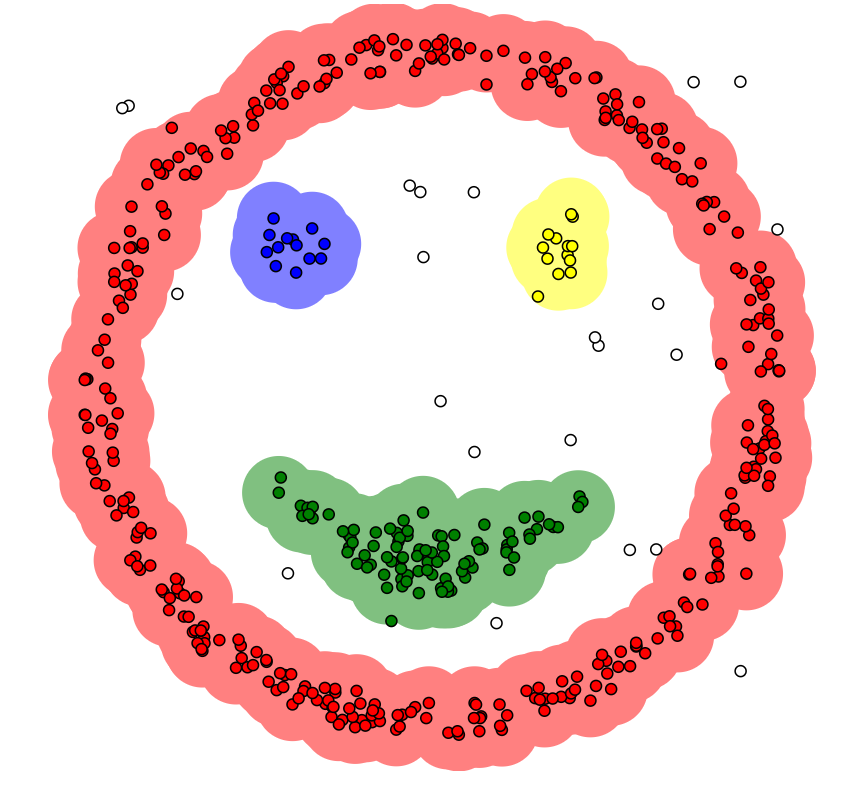3. 擅长找到离群点（还有一些没有颜色的点就是离群点，不满足epsilon = 1.00，minPoints = 4，这是上图设置的两个参数。

4. 两个参数就够了

1. 高维数据处理有些困难（理论是这样，但是博主的实力现在还做不了这种实验，但是可以从另一个角度想，高维数据，点之间稀疏，密度就很难定义）
2. 参数难以选择（参数对结果影响很大，如下图所示）
当epsilon = 1.00，minPoints = 4时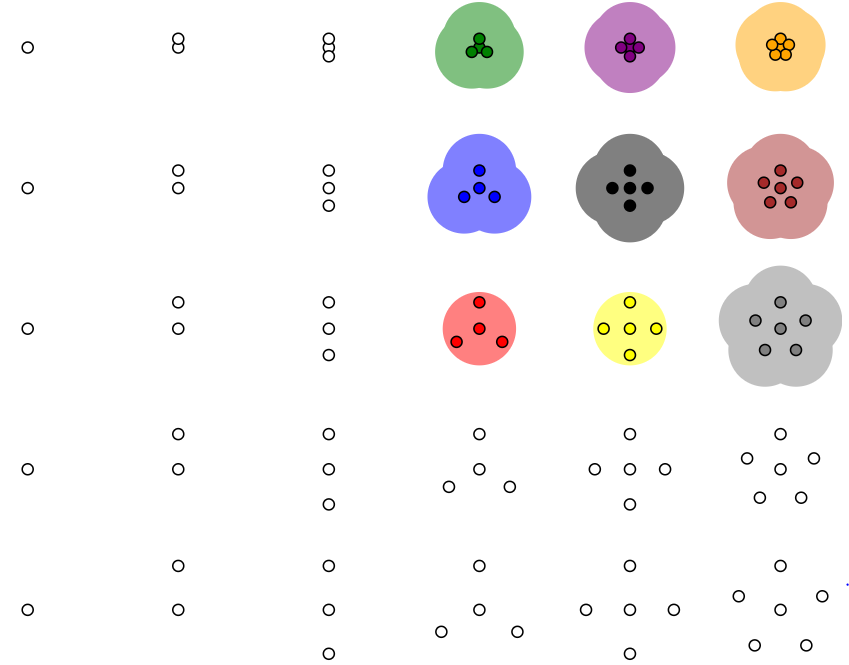当epsilon = 0.92，minPoints = 2时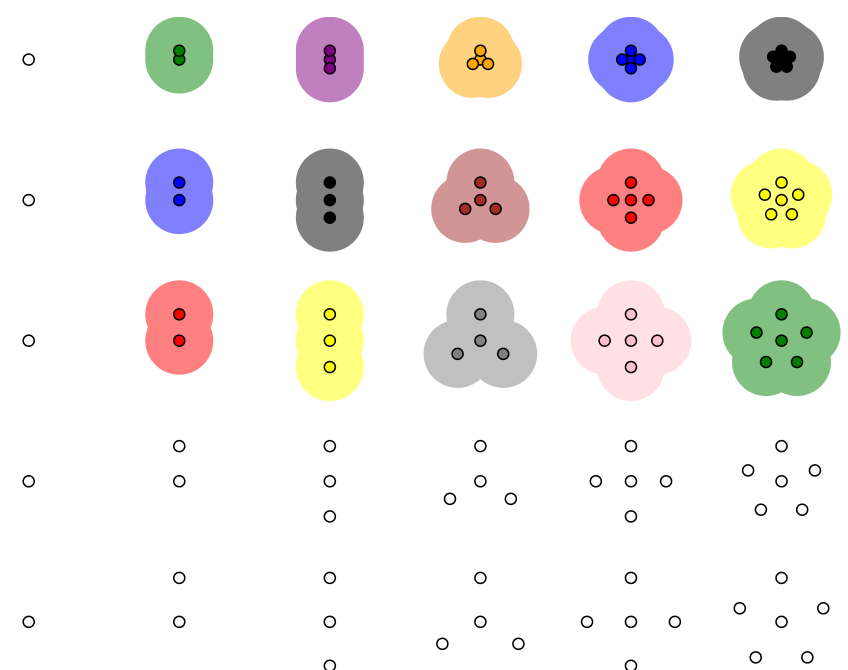#### 1.1 SciPy 聚类包

scipy.cluster是scipy下的一个做聚类的package, 共包含了两类聚类方法:

1. 矢量量化(scipy.cluster.vq):支持vector quantization 和 k-means 聚类方法
2. 层次聚类(scipy.cluster.hierarchy):支持hierarchical clustering 和 agglomerative clustering(凝聚聚类)
# coding=utf-8
from pylab import *
from scipy.cluster.vq import *

# 添加中文字体支持
from matplotlib.font_manager import FontProperties
font = FontProperties(fname=r"c:\windows\fonts\SimSun.ttc", size=14)

class1 = 1.5 * randn(100, 2)
class2 = randn(100, 2) + array([5, 5])
features = vstack((class1, class2))
centroids, variance = kmeans(features, 2)     #计算方差
code, distance = vq(features, centroids)
figure()
ndx = where(code == 0)
plot(features[ndx, 0], features[ndx, 1], '*')
ndx = where(code == 1)
plot(features[ndx, 0], features[ndx, 1], 'r.')
plot(centroids[:, 0], centroids[:, 1], 'go')

title(u'2维数据点聚类', fontproperties=font)
axis('off')
show()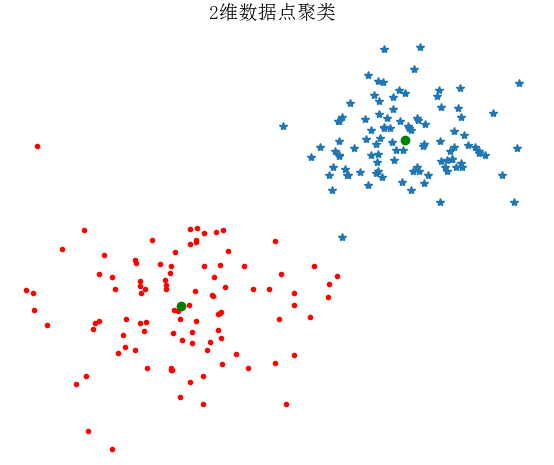#### 1.2 图像聚类

# -*- coding: utf-8 -*-
from PCV.tools import imtools
import pickle
from scipy import *
from pylab import *
from PIL import Image
from scipy.cluster.vq import *
from PCV.tools import pca

# Uses sparse pca codepath.
imlist = imtools.get_imlist('D:\\Python\\chapter6\\selectedfontimages\\a_selected_thumbs\\')

# 获取图像列表和他们的尺寸
im = array(Image.open(imlist))  # open one image to get the size
m, n = im.shape[:2]  # get the size of the images
imnbr = len(imlist)  # get the number of images
print "The number of images is %d" % imnbr

# Create matrix to store all flattened images
immatrix = array([array(Image.open(imname)).flatten() for imname in imlist], 'f')

# PCA降维
V, S, immean = pca.pca(immatrix)

# 保存均值和主成分
f = open('D:\\Python\\chapter6\\fontimages\\font_pca_modes.pkl', 'wb')
pickle.dump(immean, f)
pickle.dump(V, f)
f.close()

# 获取 selected-fontimages 文件下图像文件名，并保存在列表中
imlist = imtools.get_imlist('D:\\Python\\chapter6\\selectedfontimages\\a_selected_thumbs\\')
imnbr = len(imlist)

# 载入模型文件
with open('D:\\Python\\chapter6\\fontimages\\font_pca_modes.pkl', 'rb') as f:
# 创建矩阵，存储所有拉成一组形式后的图像
immatrix = array([array(Image.open(im)).flatten() for im in imlist], 'f')

# 投影到前 40 个主成分上
immean = immean.flatten()
projected = array([dot(V[:40], immatrix[i] - immean) for i in range(imnbr)])

# 进行 k-means 聚类
projected = whiten(projected)
centroids, distortion = kmeans(projected, 4)
code, distance = vq(projected, centroids)

# 绘制聚类簇
for k in range(4):
ind = where(code == k)
figure()
gray()
for i in range(minimum(len(ind), 40)):
subplot(4, 10, i + 1)
imshow(immatrix[ind[i]].reshape((25, 25)))
axis('off')
show()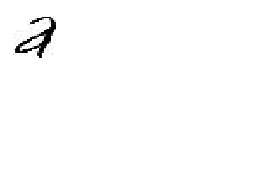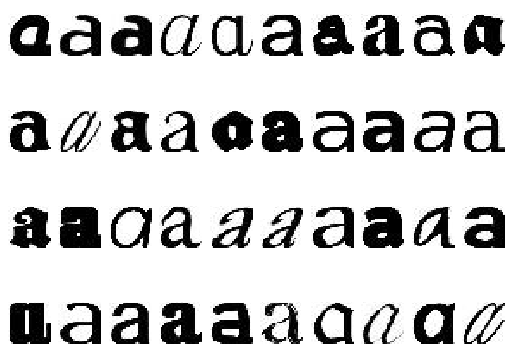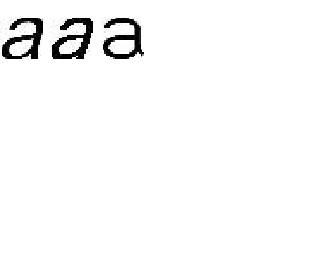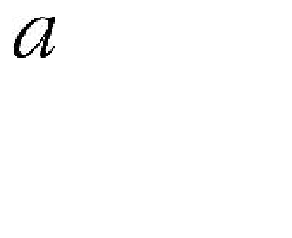#### 1.1 在主成分上可视化图像

projected = array([dot(V[[0,2]],immatrix[i]-immean) for i in range(imnbr)])

# -*- coding: utf-8 -*-
from PCV.tools import imtools, pca
from PIL import Image, ImageDraw
from pylab import *
from PCV.clustering import hcluster

imlist = imtools.get_imlist('D:\\Python\\chapter6\\selectedfontimages\\a_selected_thumbs')
imnbr = len(imlist)

immatrix = array([array(Image.open(im)).flatten() for im in imlist], 'f')
V, S, immean = pca.pca(immatrix)

# Project on 2 PCs.
projected = array([dot(V[[0, 1]], immatrix[i] - immean) for i in range(imnbr)])
# projected = array([dot(V[[1, 2]], immatrix[i] - immean) for i in range(imnbr)])

# 高和宽
h, w = 1200, 1200

# 创建一幅白色背景图
img = Image.new('RGB', (w, h), (255, 255, 255))
draw = ImageDraw.Draw(img)

# 绘制坐标轴
draw.line((0, h / 2, w, h / 2), fill=(255, 0, 0))
draw.line((w / 2, 0, w / 2, h), fill=(255, 0, 0))

# 缩放以适应坐标系
scale = abs(projected).max(0)
scaled = floor(array([(p / scale) * (w / 2 - 20, h / 2 - 20) + (w / 2, h / 2)
for p in projected])).astype(int)

# 粘贴每幅图像的缩略图到白色背景图片
for i in range(imnbr):
nodeim = Image.open(imlist[i])
nodeim.thumbnail((25, 25))
ns = nodeim.size
box = (scaled[i] - ns // 2, scaled[i] - ns // 2,
scaled[i] + ns // 2 + 1, scaled[i] + ns // 2 + 1)
img.paste(nodeim, box)

tree = hcluster.hcluster(projected)
hcluster.draw_dendrogram(tree, imlist, filename='fonts.png')

figure()
imshow(img)
axis('off')
img.save('D:\\Python\\chapter6\\pca_font.png')
show()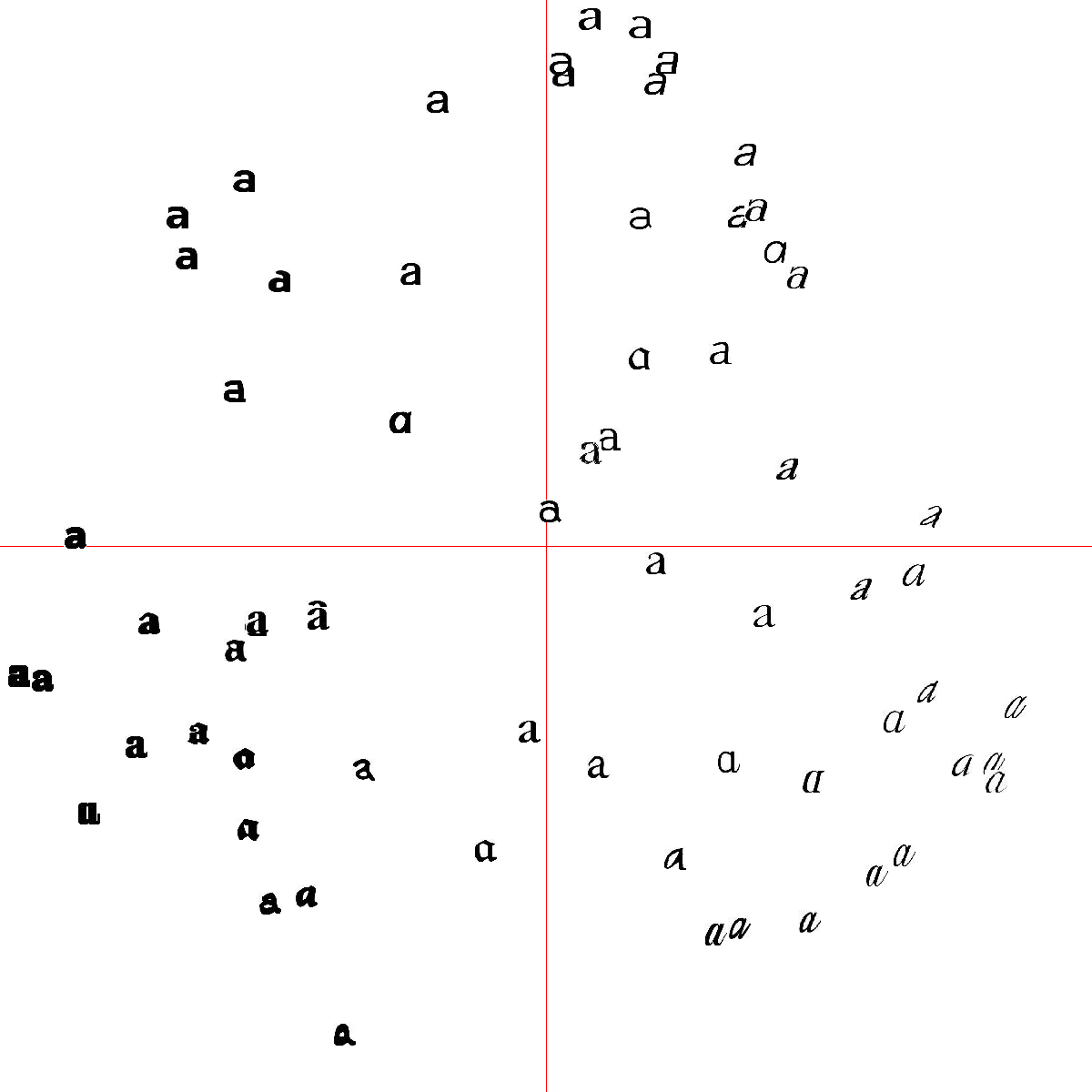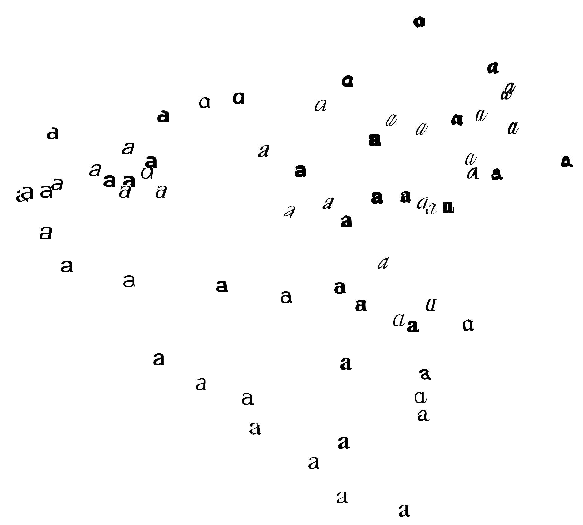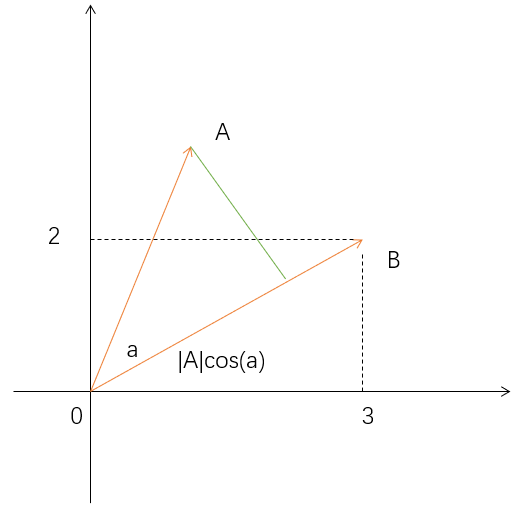B向量可以表示为(3,2)，实际上表示线性组合：$x(1,0)^{T}+y(0,1)^{T}$

：(1,0)和(0,1)叫做二维空间的一组基。

$\begin{pmatrix} 1/\sqrt{2}& 1/\sqrt{2}\\ - 1/\sqrt{2}& 1/\sqrt{2} \end{pmatrix}\binom{3}{2}=\binom{5/\sqrt{2}}{-1/\sqrt{2}}$

$\begin{pmatrix} p_{1}\\ p_{2}\\ .\\ .\\ .\\ p_{R}\end{pmatrix}\begin{pmatrix} a_{1} & a_{2}& . & .& . & a_{M} \end{pmatrix}=\begin{pmatrix} p_{1}a_{1} & p_{1}a_{2} & . &. & . &p_{1}a_{M} \\ p_{2}a_{1} & p_{2}a_{2} & . &. & . &p_{2}a_{M}\\ .& . &. & .& . & .\\ .& . &. & .& . & .\\ .& . &. & .& . & .\\ p_{R}a_{1} & p_{R}a_{2} & . &. & . &p_{R}a_{M} \end{pmatrix}$

（表示a特征和b特征之间的关系，如果a和b变化趋势相同，则它们的协方差会很大，越接近1表示a和b的变化程度越大，协方差值在-1到1之间）

$\frac{1}{m}XX^{T}=\begin{pmatrix} \frac{1}{m}\sum_{i=1}^{m}a_{i}^{2} &\frac{1}{m}\sum_{i=1}^{m}a_{i}b_{i} \\ \frac{1}{m}\sum_{i=1}^{m}a_{i}b_{i} & \frac{1}{m}\sum_{i=1}^{m}b_{i}^{2} \end{pmatrix}$

X是数据，$XX^T$相当于协方差矩阵，m是样本个数，矩阵对角线上的两个元素分别是两个字段的方差，而其它元素是a和b的协方差。

$E=\begin{pmatrix} e_{1} & e_{2} &... & e_{n} \end{pmatrix}$
$E^{T}CE=\Lambda= \begin{pmatrix} \lambda _{1} & & & & & \\ & \lambda 2 & & & & \\ & & . & & & \\ & & &. & & \\ & & & & . & \\ & & & & & \lambda n \end{pmatrix}$

pca在数据分析中的应用

from sklearn.preprocessing import StandardScaler
import numpy as np
import pandas as pd
X = df.ix[:,0:4].values
X_std = StandardScaler().fit_transform(X)
print (X_std)     #这是一个四维数据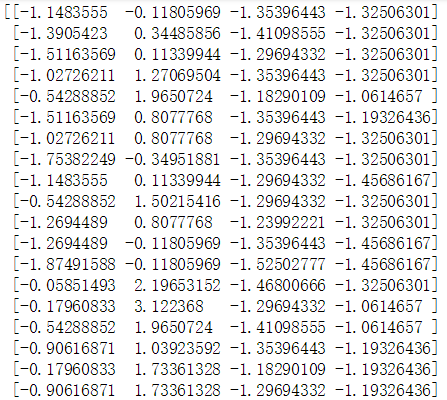mean_vec = np.mean(X_std, axis=0)
cov_mat = (X_std - mean_vec).T.dot((X_std - mean_vec)) / (X_std.shape-1)   #对应下面公式
print('Covariance matrix \n%s' %cov_mat)  #输出协方差矩阵


$\sum =\frac{1}{n-1}((X-\bar{x})^{T}(X-\bar{x}))$

$\bar{x}=\frac{1}{n}\sum_{k=1}^{n}x_{i}$

Covariance matrix
[[ 1.00675676 -0.10448539 0.87716999 0.82249094]
[-0.10448539 1.00675676 -0.41802325 -0.35310295]
[ 0.87716999 -0.41802325 1.00675676 0.96881642]
[ 0.82249094 -0.35310295 0.96881642 1.00675676]]

cov_mat = np.cov(X_std.T)

eig_vals, eig_vecs = np.linalg.eig(cov_mat)

print('Eigenvectors \n%s' %eig_vecs)    #特征向量
print('\nEigenvalues \n%s' %eig_vals)    #特征值


Eigenvectors
[[ 0.52308496 -0.36956962 -0.72154279 0.26301409]
[-0.25956935 -0.92681168 0.2411952 -0.12437342]
[ 0.58184289 -0.01912775 0.13962963 -0.80099722]
[ 0.56609604 -0.06381646 0.63380158 0.52321917]]

Eigenvalues
[ 2.92442837 0.93215233 0.14946373 0.02098259]

tot = sum(eig_vals)
var_exp = [(i / tot)*100 for i in sorted(eig_vals, reverse=True)]      #归一化操作，把特征值映射成一个百分数
print (var_exp)
cum_var_exp = np.cumsum(var_exp)  #后一个值加前面一个值
cum_var_exp


[72.620033326920336, 23.147406858644135, 3.7115155645845164, 0.52104424985101538] #归一化后的结果，变成百分数，例如第一个值占了72%重要
array([ 72.62003333, 95.76744019, 99.47895575, 100. ])

plt.figure(figsize=(6, 4))

plt.bar(range(4), var_exp, alpha=0.5, align='center',
label='individual explained variance')
plt.step(range(4), cum_var_exp, where='mid',
label='cumulative explained variance')
plt.ylabel('Explained variance ratio')
plt.xlabel('Principal components')
plt.legend(loc='best')
plt.tight_layout()
plt.show()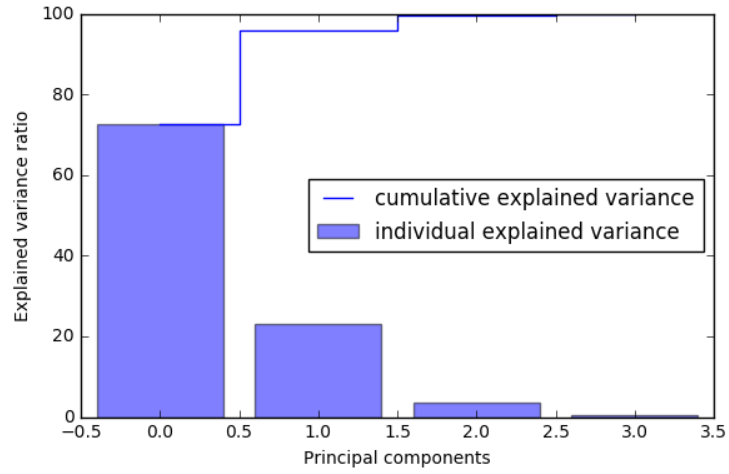matrix_w = np.hstack((eig_pairs.reshape(4,1),
eig_pairs.reshape(4,1)))   #先找第一大特征向量，再找第二大特征向量，组合到一块，成为4x2矩阵

print('Matrix W:\n', matrix_w)   #变换矩阵


Matrix W:
[[ 0.52308496 -0.36956962]
[-0.25956935 -0.92681168]
[ 0.58184289 -0.01912775]
[ 0.56609604 -0.06381646]]

Y = X_std.dot(matrix_w)   #最终得到150x2矩阵
Y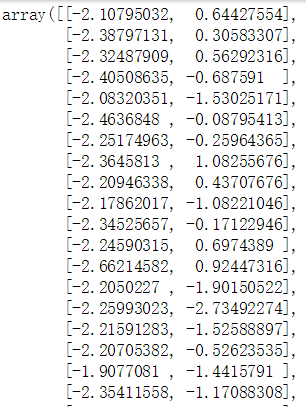plt.figure(figsize=(6, 4))
for lab, col in zip(('Iris-setosa', 'Iris-versicolor', 'Iris-virginica'),
('blue', 'red', 'green')):
plt.scatter(X[y==lab, 0],
X[y==lab, 1],
label=lab,
c=col)
plt.xlabel('sepal_len')
plt.ylabel('sepal_wid')
plt.legend(loc='best')
plt.tight_layout()
plt.show()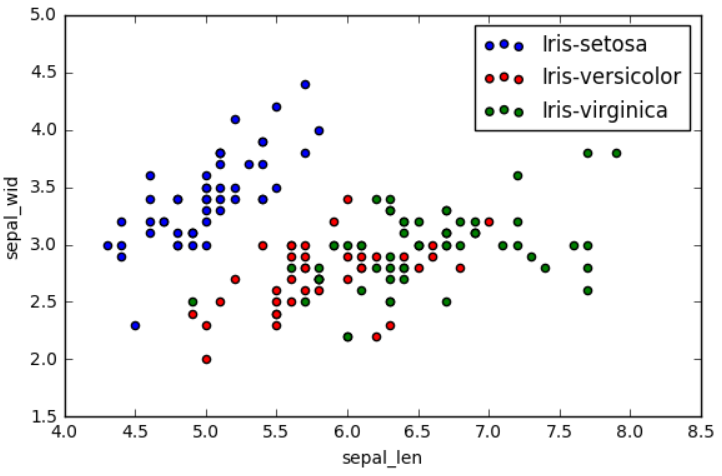plt.figure(figsize=(6, 4))
for lab, col in zip(('Iris-setosa', 'Iris-versicolor', 'Iris-virginica'),
('blue', 'red', 'green')):
plt.scatter(Y[y==lab, 0],
Y[y==lab, 1],
label=lab,
c=col)
plt.xlabel('Principal Component 1')
plt.ylabel('Principal Component 2')
plt.legend(loc='lower center')
plt.tight_layout()
plt.show()    #四维变换成两维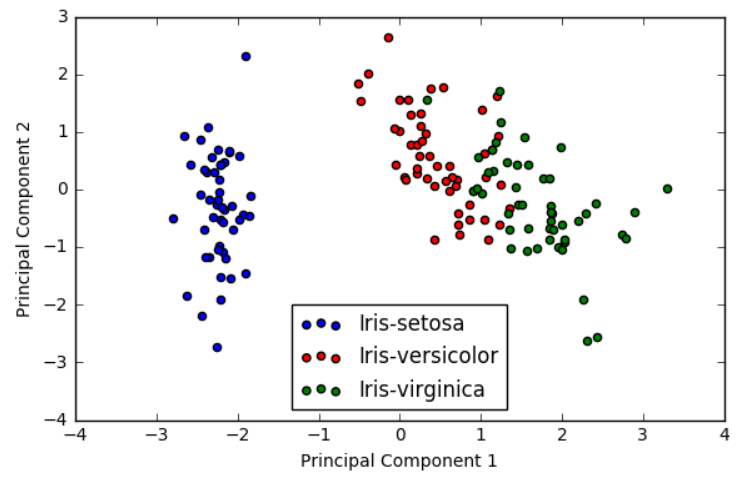#### 1.1 像素聚类

# -*- coding: utf-8 -*-

from scipy.cluster.vq import *
from scipy.misc import imresize
from pylab import *
from PIL import Image, ImageFont

# 添加中文字体支持
from matplotlib.font_manager import FontProperties

font = FontProperties(fname=r"c:\windows\fonts\SimSun.ttc", size=14)

def clusterpixels(infile, k, steps):
im = array(Image.open(infile))
dx = im.shape / steps
dy = im.shape / steps
# compute color features for each region
features = []
for x in range(steps):
for y in range(steps):
R = mean(im[x * dx:(x + 1) * dx, y * dy:(y + 1) * dy, 0])
G = mean(im[x * dx:(x + 1) * dx, y * dy:(y + 1) * dy, 1])
B = mean(im[x * dx:(x + 1) * dx, y * dy:(y + 1) * dy, 2])
features.append([R, G, B])
features = array(features, 'f')  # make into array
# 聚类， k是聚类数目
centroids, variance = kmeans(features, k)
code, distance = vq(features, centroids)
# create image with cluster labels
codeim = code.reshape(steps, steps)
codeim = imresize(codeim, im.shape[:2], 'nearest')
return codeim

k = 3
infile_empire = 'D:\\Python\\chapter6\\empire.jpg'
im_empire = array(Image.open(infile_empire))
infile_boy_on_hill = 'D:\\Python\\chapter6\\boy_on_hill.jpg'
im_boy_on_hill = array(Image.open(infile_boy_on_hill))
steps = (50, 100)  # image is divided in steps*steps region
print steps, steps[-1]

# 显示原图empire.jpg
figure()
subplot(231)
title(u'原图', fontproperties=font)
axis('off')
imshow(im_empire)

# 用50*50的块对empire.jpg的像素进行聚类
codeim = clusterpixels(infile_empire, k, steps)
subplot(232)
title(u'k=3,steps=50', fontproperties=font)
# ax1.set_title('Image')
axis('off')
imshow(codeim)

# 用100*100的块对empire.jpg的像素进行聚类
codeim = clusterpixels(infile_empire, k, steps[-1])
ax1 = subplot(233)
title(u'k=3,steps=100', fontproperties=font)
# ax1.set_title('Image')
axis('off')
imshow(codeim)

# 显示原图empire.jpg
subplot(234)
title(u'原图', fontproperties=font)
axis('off')
imshow(im_boy_on_hill)

# 用50*50的窗口对empire.jpg的像素进行聚类
codeim = clusterpixels(infile_boy_on_hill, k, steps)
subplot(235)
title(u'k=3,steps=50', fontproperties=font)
# ax1.set_title('Image')
axis('off')
imshow(codeim)

# 用100*100的窗口对empire.jpg的像素进行聚类
codeim = clusterpixels(infile_boy_on_hill, k, steps[-1])
subplot(236)
title(u'k=3，steps=100', fontproperties=font)
axis('off')
imshow(codeim)

show()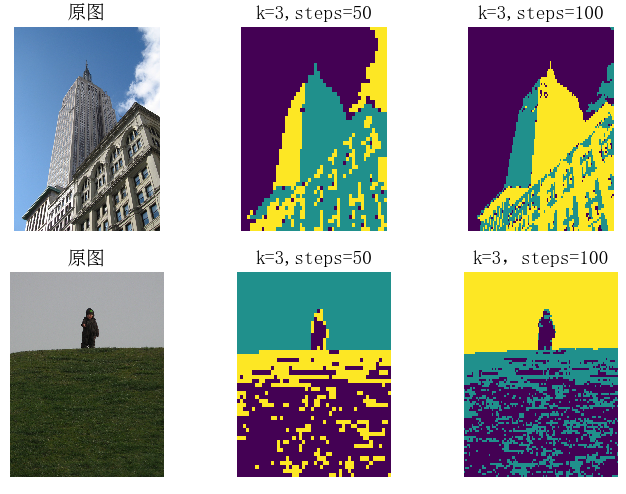K-means 的输入是一个有 steps×steps 行的数组，数组的每一行有 3 列，各列分别为区域块 R、G、B 三个通道的像素平均值。为可视化最后的结果 , 用 SciPy 的 imresize() 函数在原图像坐标中显示这幅 steps×steps 的图像。参数 interp 指定插值方法；采用最近邻插值法，以便在类间进行变换时不需要引入新的像素值。K-means 标签的次序是任意的（在这里的标签指最终结果中图像的颜色）。正如看到的，尽管利用窗口对它进行了下采样，但结果仍然是有噪声的。如果图像某些区域没有空间一致性，则很难将它们分开，如图中小男孩和草坪的图。

## （二）层次聚类

1. 可以通过绘制树状图(dendrogram)，帮助我们使用可视化的方式来解释聚类结果。
2. 不需要事先指定簇的数量。

1. 获取所有样本的距离矩阵
2. 将每个数据点作为一个单独的簇
3. 基于最不相似(距离最远)样本的距离，合并两个最接近的簇
4. 更新样本的距离矩阵
5. 重复2到4，直到所有样本都属于同一个簇为止。

1.获取样本，随机产生5个样本，每个样本包含3个特征(x、y、z)。

import pandas as pd
import numpy as np

if __name__ == "__main__":
np.random.seed(1)
#设置特征的名称
variables = ["x","y","z"]
#设置编号
labels = ["s1","s2","s3","s4","s5","s6"]
#产生一个(6,3)的数组
data = np.random.random_sample([6,3])*10
#通过pandas将数组转换成一个DataFrame
df = pd.DataFrame(data,columns=variables,index=labels)
#查看数据
print(df)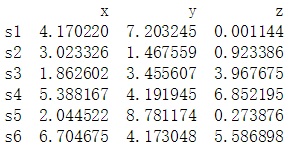2.获取所有样本的距离矩阵，通过SciPy来计算距离矩阵，计算每个样本间两两的欧式距离，将矩阵矩阵用一个DataFrame进行保存，方便查看。

    from scipy.spatial.distance import pdist,squareform
#获取距离矩阵
'''
pdist:计算两两样本间的欧式距离,返回的是一个一维数组
squareform：将数组转成一个对称矩阵
'''
dist_matrix = pd.DataFrame(squareform(pdist(df,metric="euclidean")),
columns=labels,index=labels)
print(dist_matrix)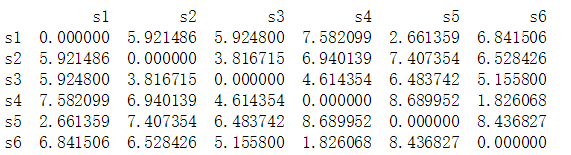from scipy.cluster.hierarchy import linkage
#以全连接作为距离判断标准，获取一个关联矩阵
#将关联矩阵转换成为一个DataFrame
clusters = pd.DataFrame(row_clusters,columns=["label 1","label 2","distance","sample size"],
index=["cluster %d"%(i+1) for i in range(row_clusters.shape)])
print(clusters)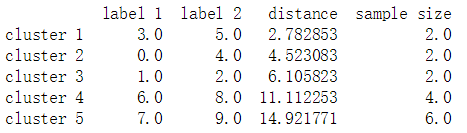4.通过关联矩阵绘制树状图，使用scipy的dendrogram来绘制树状图。

    from scipy.cluster.hierarchy import dendrogram
import matplotlib.pyplot as plt
row_dendr = dendrogram(row_clusters,labels=labels)
plt.tight_layout()
plt.ylabel("欧式距离")
plt.show()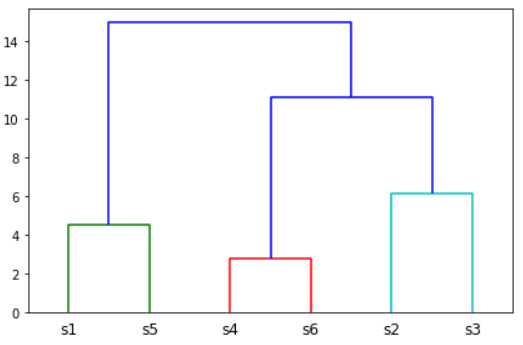# -*- coding: utf-8 -*-
import os
from PCV.clustering import hcluster
from matplotlib.pyplot import *
from numpy import *
from PIL import Image

# 创建图像列表
path = 'D:\\Python\\chapter6\\sunsets\\flickr-sunsets-small\\'
imlist = [os.path.join(path, f) for f in os.listdir(path) if f.endswith('.jpg')]
# 提取特征向量，每个颜色通道量化成 8 个小区间
features = zeros([len(imlist), 512])
for i, f in enumerate(imlist):
im = array(Image.open(f))
# 多维直方图
h, edges = histogramdd(im.reshape(-1, 3), 8, normed=True, range=[(0, 255), (0, 255), (0, 255)])
features[i] = h.flatten()
tree = hcluster.hcluster(features)

# 设置一些（任意的）阈值以可视化聚类簇
clusters = tree.extract_clusters(0.23 * tree.distance)
# 绘制聚类簇中元素超过 3 个的那些图像
for c in clusters:
elements = c.get_cluster_elements()
nbr_elements = len(elements)
if nbr_elements > 3:
figure()
for p in range(minimum(nbr_elements, 20)):
subplot(4, 5, p + 1)
im = array(Image.open(imlist[elements[p]]))
imshow(im)
axis('off')
show()

hcluster.draw_dendrogram(tree, imlist, filename='sunset.pdf')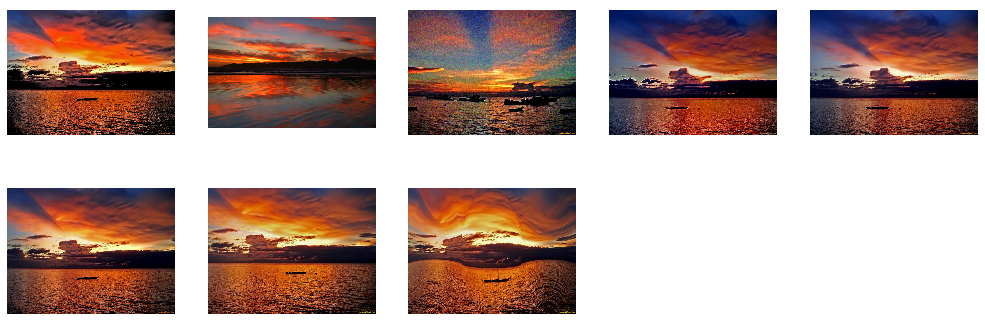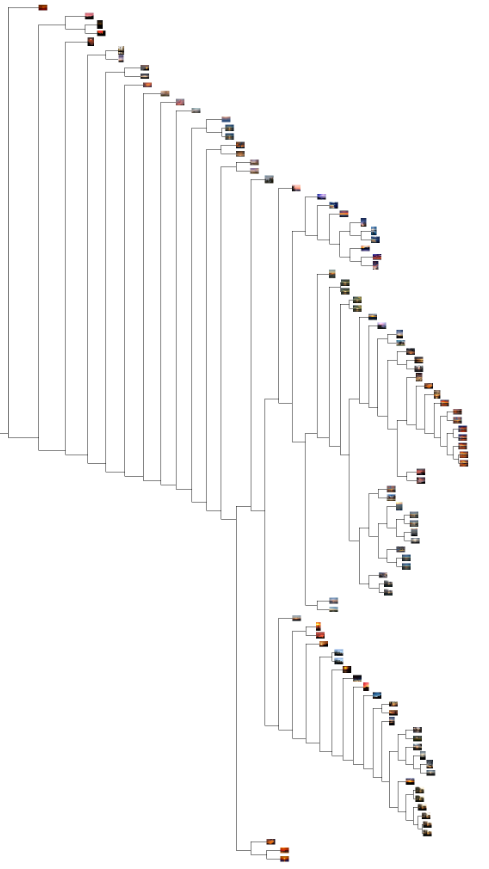tree = hcluster.hcluster(projected) hcluster.draw_dendrogram(tree,imlist,filename='fonts.jpg')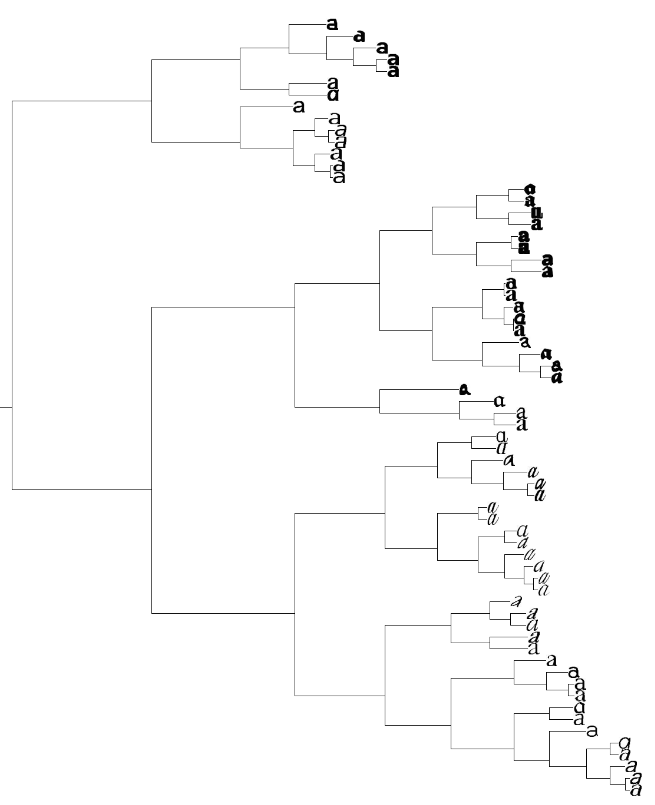## （三）谱聚类

：由若干及连接两点的线所构成的图像，通常用来描述某些事物之间的某种关系，用点代表事物，线表示对应两个事物间具有的关系。（有箭头是有向图，没有箭头是无向图，这个离散数学应该学过）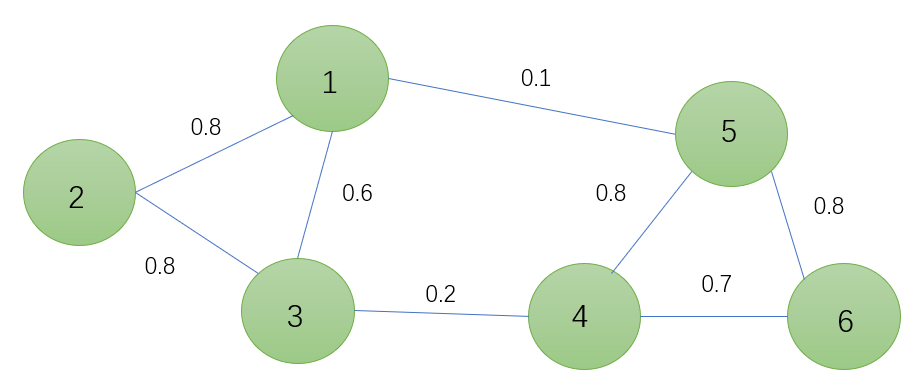$G(V,E)$表示无向图（蓝色的线没有箭头），$V=\left \{ v_{1},v_{2},...,v_{n} \right \}$表示点集（1,2,3,4,5,6这些点），E表示边集(蓝色的线)。

$W_{ij}$表示$V_i$$V_j$之间的关系，称作权重（1到5之间的权重为0.1，5到1的权重也为0.1），对于无向图，$W_{ij}=W_{ji}$而且$W_{ii}=0$ (1到1本身权重为0) ， $W_{ij}>0$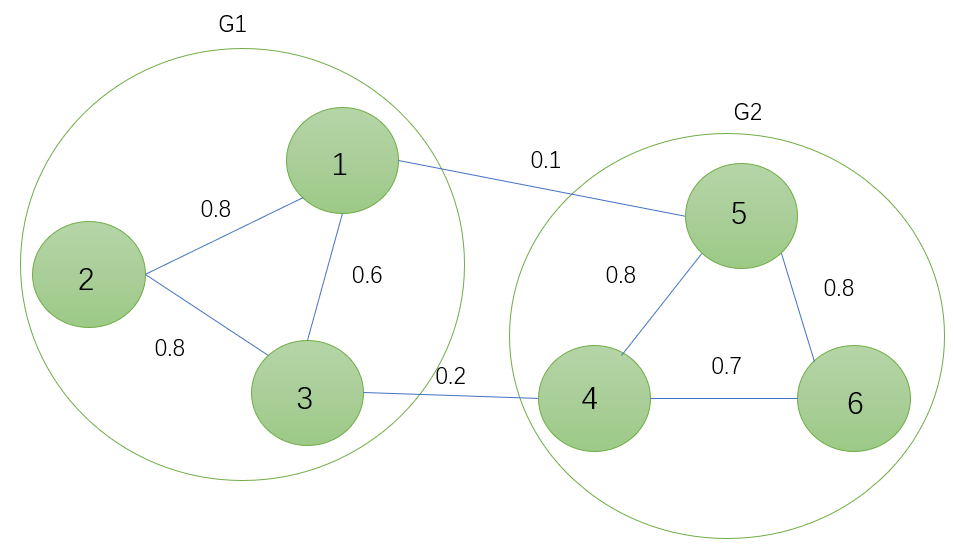$Cut(G_{1},G_{2})=\sum_{i\epsilon G_{1},j\epsilon G_{2}}^{}W_{ij}$

1，2，3聚类为$G_{1}$，4，5，6聚类为$G_{2}$，中间的权重0.1,0.2就是$W_{ij}$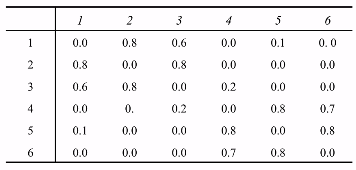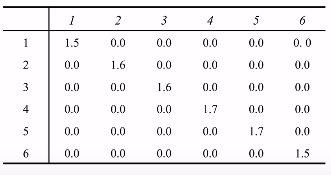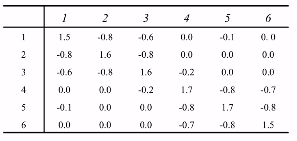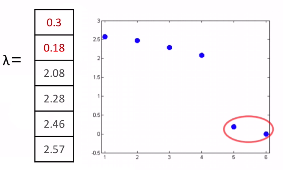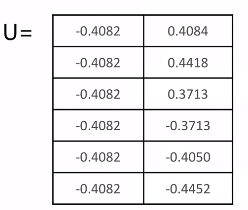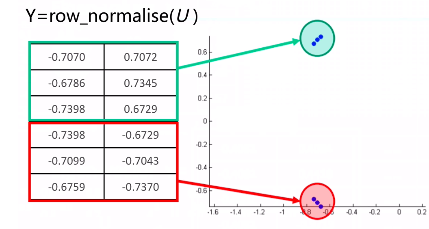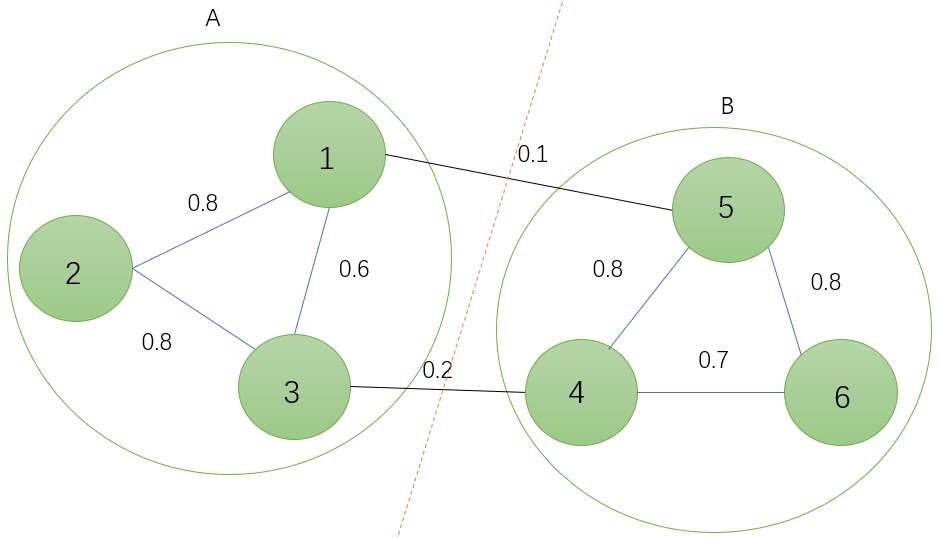1. 数据准备，生成图的邻接矩阵
2. 归一化拉普拉斯矩阵
3. 生成最小的K个特征值和对应的特征向量
4. 将特征向量kmeans聚类

1. 处理稀疏数据（谱聚类只需要数据之间的邻接矩阵，因此对于处理稀疏数据的聚类很有效）
2. 处理高维数据（使用了降维，因此在处理高维数据聚类时的复杂度比传统聚类算法好，在高维数据上表现明显）

1. 如果最终聚类的维度非常高，则由于降维的幅度不够，谱聚类的运行速度和最后的聚类效果均不好。
2. 最终效果可能不同（聚类效果依赖于邻接矩阵，不同的邻接矩阵得到的最终聚类效果可能有很大不同）

# -*- coding: utf-8 -*-
from PCV.tools import imtools, pca
from PIL import Image, ImageDraw
from pylab import *
from scipy.cluster.vq import *

imlist = imtools.get_imlist('D:\\Python\\chapter6\\selectedfontimages\\a_selected_thumbs')
imnbr = len(imlist)

immatrix = array([array(Image.open(im)).flatten() for im in imlist], 'f')
V, S, immean = pca.pca(immatrix)

# Project on 2 PCs.
projected = array([dot(V[[0, 1]], immatrix[i] - immean) for i in range(imnbr)])  # P131 Fig6-3左图
# projected = array([dot(V[[1, 2]], immatrix[i] - immean) for i in range(imnbr)])  # P131 Fig6-3右图

n = len(projected)
# 计算距离矩阵
S = array([[sqrt(sum((projected[i] - projected[j]) ** 2))
for i in range(n)] for j in range(n)], 'f')
# 创建拉普拉斯矩阵
rowsum = sum(S, axis=0)
D = diag(1 / sqrt(rowsum))
I = identity(n)
L = I - dot(D, dot(S, D))
# 计算矩阵 L 的特征向量
U, sigma, V = linalg.svd(L)
k = 5
# 从矩阵 L 的前k个特征向量（eigenvector）中创建特征向量（feature vector） # 叠加特征向量作为数组的列
features = array(V[:k]).T
# k-means 聚类
features = whiten(features)
centroids, distortion = kmeans(features, k)
code, distance = vq(features, centroids)
# 绘制聚类簇
for c in range(k):
ind = where(code == c)
figure()
gray()
for i in range(minimum(len(ind), 39)):
im = Image.open(imlist[ind[i]])
subplot(4, 10, i + 1)
imshow(array(im))
axis('equal')
axis('off')
show()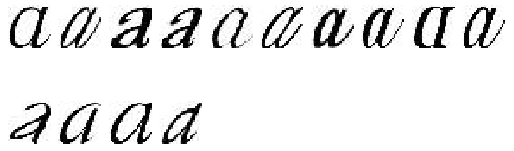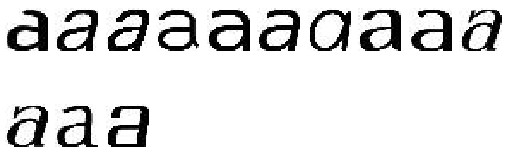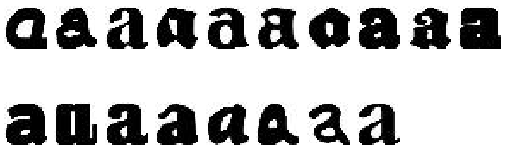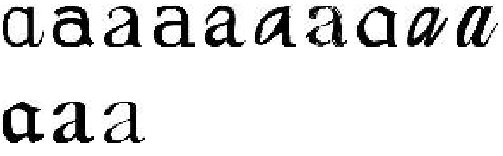©️2019 CSDN 皮肤主题: 技术工厂 设计师: CSDN官方博客